• ## 带约束的最优化问题

万次阅读 多人点赞 2016-11-01 01:12:13
学习支持向量机SVM时，拉格朗日对偶、Slater条件、KKT条件等大堆概念... 为什么要引入堆系数把约束条件与目标函数组合到一起？为什么满足了KKT条件就是符合条件的解? 为什么可以转化为对偶问题？什么是Slater条件？
支持向量机SVM的训练过程中需要求解带约束的最优化问题。

带约束的最优化问题通常表述如下：

objective:minw f(w)s.t. {gi(w)≤0,hj(w)=0,i=1,2,3...n;j=1,2,3...m;不等式约束等式约束

o

b

j

e

c

t

i

v

e

:

min

w

f

(

w

)

s

.

t

.

{

g

i

(

w

)

≤

0

,

i

=

1

,

2

,

3...

n

;

不等式约束

h

j

(

w

)

=

0

,

j

=

1

,

2

,

3...

m

;

等式约束

s.t.\
\begin{cases}
g_i(w)\le0, &i=1,2,3...n;&\text{不等式约束}\\
h_j(w)=0,&j=1,2,3...m;&\text{等式约束}
\end{cases}

gi(w)

g

i

(

w

)

$g_i(w)$,

hj(w)

h

j

(

w

)

$h_j(w)$和

f(w)

f

(

w

)

$f(w)$一般都是连续可导的。要求在满足

gi(w)≤0,hj(w)=0

g

i

(

w

)

≤

0

,

h

j

(

w

)

=

0

$g_i(w)\le0,h_j(w)=0$的前提下求能使得目标函数

f(w)

f

(

w

)

$f(w)$最小化的

w

w

$w$。

学习支持向量机SVM时，拉格朗日对偶、Slater条件、KKT条件等一大堆概念席卷而来，让没系统学习过凸优化的笔者一头雾水，估计不少人都是这样吧。
不幸的是笔者在数学相关问题上经常一不小心就陷入死磕，遇到没想明白的问题就总惦记着。另外笔者还是个懒于动手的人，但好在数学是一门完全不需要动手的学科，你只要靠想就可以，最多需要在纸上画画，完全不用做什么实验或者手工体力活儿，嗯，很适合笔者这种懒人，这也是笔者为什么从一个电气工程学生慢慢偏离航向发展成一个程序猿的原因。
回到我们要说的SVM中的优化问题。为什么要引入一堆系数把约束条件与目标函数组合到一起？为什么满足了KKT条件就是符合条件的解? 为什么可以转化为对偶问题？什么是Slater条件？在讲SVM的资料中通常不会详细展开这些问题，只是告知读者有这么回事。带着一排排问号，翻阅文献、Wiki、博客，加上自己的一些思考，算是把不少问题整理清楚了。笔者希望能把这些问题用容易理解的方式讲述明白，通过这一系列博文，将相关问题由易到难一一做出解释，希望这个系列的文章能帮更多人解惑。

本系列文章包括：
1. 纯等式约束时的optimalize问题
2. 纯不等式约束时的optimalize问题
3.混合约束时的optimalize问题——KKT条件与拉格朗日对偶问题
4.强对偶性的证明与Slater条件

1. 纯等式约束时的optimalize问题

这一类问题的范式如下：

在满足

hj(w)=0

h

j

(

w

)

=

0

$h_j(w)=0$的条件下使

f(w)

f

(

w

)

$f(w)$最小化。

hj(w)

h

j

(

w

)

$h_j(w)$和

f(w)

f

(

w

)

$f(w)$都应是连续可导的。上面纯代数的形式会让人不容易看到此类问题的本质，我们希望对这类问题有一个直观的几何理解。首先我们来看一下每个等式约束的含义。
等式约束的几何意义
对于

hj(w)=0

h

j

(

w

)

=

0

$h_j(w)=0$，如果

w

w

$w$是一个3维变量，那所有满足hj(w)=0${h}_{j}\left(w\right)=0$$h_j(w)=0$的

w

w

$w$点集通常会组成一个 2 维的曲面。特别当hj(w)${h}_{j}\left(w\right)$$h_j(w)$是

w

w

$w$的线性函数时，比如 ：
hj(w)=a1wx+a2wy+a3wz+b${h}_{j}\left(w\right)={a}_{1}{w}_{x}+{a}_{2}{w}_{y}+{a}_{3}{w}_{z}+b$h_j(w)=a_1w_x+a_2w_y+a_3w_z+b  其中

wx、wy、wz

w

x

、

w

y

、

w

z

$w_x、w_y、w_z$分别是

w

$w$在三个维度上的分量，${a}_{1}、{a}_{2}、{a}_{3}、b$$a_1、a_2、a_3、b$是某些常数。这时

h

j

(

w

)

=

0

$h_j(w)=0$就是三维空间中的一个平面。对于通常的非线性函数而言，

h

j

(

w

)

=

0

$h_j(w)=0$一般对应一个曲面。    如果

w

$w$是更高维的变量，维数设为${d}_{w}$$d_w$，那

h

j

(

w

)

=

0

$h_j(w)=0$一般对应一个

(

d

w

−

1

)

$(d_w-1)$维的子空间，或者说

(

d

w

−

1

)

$(d_w-1)$维的曲面。注意，当我们下面再提到”面”这个概念时，它不一定代表狭义的二维面，而可以是对任意维度的一个子空间的称谓。  借用下物理中的概念：如果将

h

j

(

w

)

$h_j(w)$看做空间中某个向量场的势函数，这个向量场就是

h

j

(

w

)

$h_j(w)$的梯度场

∇

h

j

(

w

)

$\nabla h_j(w)$，而

h

j

(

w

)

=

0

$h_j(w)=0$所对应的子空间就是该梯度场的0等势面。在等势面上任一点的梯度都与该点邻域内的等势面垂直，即若

w

∗

$w^*$是等势面上一点，则

∇

h

j

(

w

∗

)

$\nabla h_j(w^*)$垂直于该等势面。（通常等势面是个曲面，当我们说垂直的时候，只是针对该面上某个点的切面）  进一步的，

c

∇

h

j

(

w

∗

)

$c\nabla h_j(w^*)$构成了一个1维的子空间

G

j

$G_j$，这个子空间与

(

d

w

−

1

)

$(d_w-1)$维的0等势面（在

w

∗

$w^*$处的切面）垂直，因此它们互为补空间（相互垂直且维度之和是

d

w

$d_w$）。这意味着，在

w

∗

$w^*$处所有与0等势面垂直的向量都在

G

j

$G_j$子空间中，即都可表示成一个常数与

∇

h

j

(

w

∗

)

$\nabla h_j(w^*)$相乘。  继续往下读之前先确保理解了上面几段的内容。提示：在本文中遇到高维相关结论，可以先在大脑里以我们熟悉的三维空间举例理解。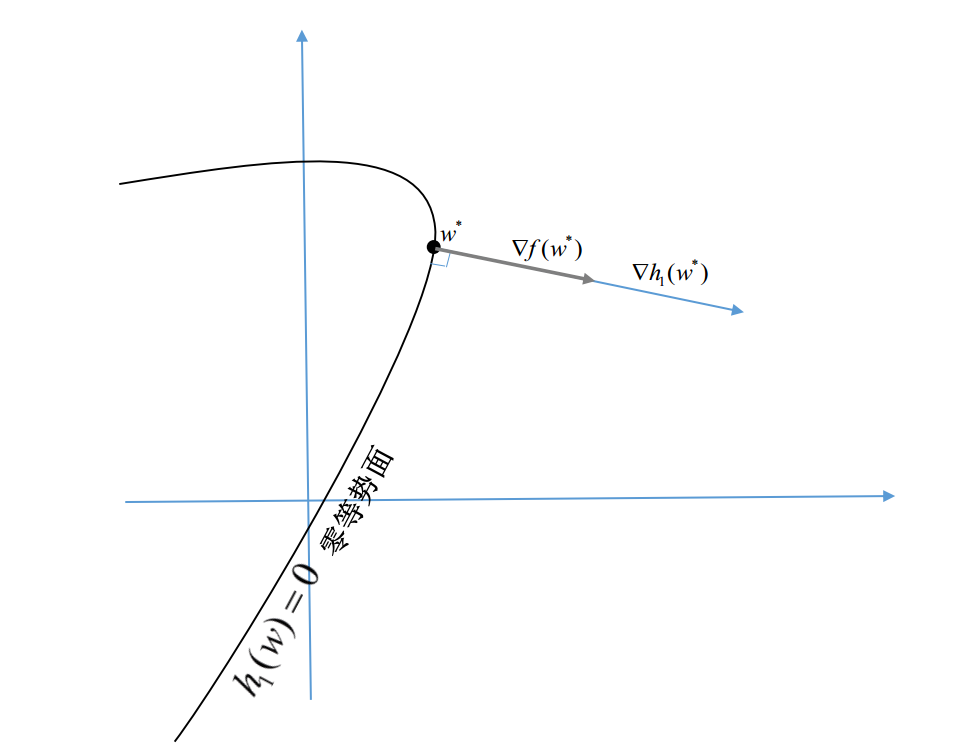再回到我们的问题，这里我们有m个等式约束，每个约束都对应一个0等势面，m个约束同时作用，相当于把可行域限制在了这m个0等势面的交集上。这个交集是什么样子？它也是一个子空间，只不过维数可能更低。比如三维空间中两个不平行的面(2维)的交集，是一条1维的线。我们先称呼前面提到的这个交集为”公共0势面”。  假设公共0势面的维度是

d

0

$d_0$，通常

d

0

=

d

w

−

m

$d_0=d_w-m$。而各

h

j

(

w

)

$h_j(w)$在公共0势面上某点

w

∗

$w^*$处的梯度向量

∇

h

j

(

w

∗

)

$\nabla h_j(w^*)$都与公共0势面垂直，且共有m个这样的向量，因此这些梯度向量组合成的m维子空间

G

$G$与公共0势面互补。意味着：${w}^{\ast }$$w^*$处所有与公共0势面垂直的向量都在

G

$G$子空间中,即都可表示成：

$V=\sum _{j=1}^{m}{c}_{j}\mathrm{\nabla }{h}_{j}\left({w}^{\ast }\right)$V=\sum_{j=1}^{m}{c_j\nabla h_j(w^*)}

当然，

d

0

$d_0$也有大于

d

w

−

m

$d_w-m$的时候，但这并不影响上面的结论。假如m个等势面中有某

(

t

+

1

)

$(t+1)$个等势面是相切的，这时公共等势面变成了

d

w

−

(

m

−

t

)

$d_w-(m-t)$维，比正常时候多了t个维度；但另一方面，梯度空间G也会因此而减少t个维度，因为有

(

t

+

1

)

$(t+1)$个梯度是平行的，它们只能提供1个维度。此时，上面的结论保持不变

公共0势面上的极值条件
我们已经知道，满足所有等式约束的点，都在前面提到的公共0势面上。那我们的目标函数

f

(

w

)

$f(w)$的极值点怎么找呢？  通常，在没有约束的时候，我们要找

f

(

w

)

$f(w)$的极值点，只要找

f

(

w

)

$f(w)$梯度为0的点，即

∇

f

(

w

)

$\nabla f(w)$是0。而我们把可行域限制在公共0势面上之后呢？对，我们只要要求梯度向量

∇

f

(

w

)

$\nabla f(w)$在公共0势面上的分量为0即可，换句话说，梯度向量与公共0势面垂直。  为了表达具体一点我们不得不把倒霉的

w

∗

$w^*$再抓回来当我们的小白鼠。我们继续把它在公共0势面上，看一下它怎样才能变成极值点。  我们要求

f

(

w

)

$f(w)$在

w

∗

$w^*$处的梯度向量

∇

f

(

w

∗

)

$\nabla f(w^*)$与该处的公共0势面垂直，意味着

∇

f

(

w

∗

)

$\nabla f(w^*)$必然在前面提到的梯度子空间G上，即它可以由各个等式约束函数在

w

∗

$w^*$处的梯度线性组合而成，即存在一系列系数

β

j

$\beta_j$，满足下式：

∇

f

(

w

∗

)

+

∑

j

=

1

m

β

j

∇

h

j

(

w

∗

)

=

0

\nabla f(w^*)+\sum_{j=1}^{m}{\beta_j\nabla h_j(w^*)}=0
这就是判定

w

∗

$w^*$是否是可行域内极值点的方法。

结论
当我们求解纯等式约束下的最优化问题时，我们只需求解如下方程组即可求得候选解，然后从中挑选最优解：

求

解

w

和

β

{

∇

f

(

w

)

+

∑

j

=

1

m

β

j

∇

h

j

(

w

)

=

0

h

j

(

w

)

=

0

f

o

r

j

=

1

,

2...

m

求解w和\beta\begin{cases}
\nabla f(w)+ \sum_{j=1}^{m} {\beta_j\nabla h_j(w)}=0\\
\end{cases}
或者我们可以构造一个能把约束条件与目标函数融合到一起的新函数：

L

(

w

,

β

)

=

f

(

w

)

+

∑

j

=

1

m

β

j

h

j

(

w

)

L(w,\beta)=f(w)+\sum_{j=1}^{m}{\beta_jh_j(w)}
不错，狡猾的笔者偷偷把
拉格朗日乘子式的雏形列了出来，就在读者察觉到怎么读了这么久还没看到拉格朗日大人的踪影是不是走错片场了的时候。
这时，上面的方程组其实就是

求

解

w

和

β

{

∂

L

∂

w

k

=

0

f

o

r

k

=

1

,

2

,

3...

d

w

(

w

的

维

数

)

∂

L

∂

β

j

=

0

f

o

r

j

=

1

,

2...

m

求解w和\beta\begin{cases}
\frac{\partial L}{\partial w_k}=0&for\ k=1,2,3...d_w(w的维数)\\
\frac{\partial L}{\partial \beta_j}=0&for\ j=1,2...m
\end{cases}
多说两句，这个方程组中共有

(

d

w

+

m

)

$(d_w+m)$ 个未知量和方程。
其中

∂

L

∂

w

k

=

0

$\frac{\partial L}{\partial w_k}=0$是同时包含

w

,

β

$w,\beta$的方程，这样的方程共有

d

w

$d_w$个;
而

∂

L

∂

β

j

=

h

j

(

w

)

=

0

$\frac{\partial L}{\partial \beta_j}=h_j(w)=0$是只包含

w

$w$的方程，这样的方程共有m个。为使$w$$w$有解，m应小于

w

$w$的维数${d}_{w}$$d_w$，否则对

w

$w$的约束方程数将大于$w$$w$的变量个数导致约束太强、各约束方程无法同时被满足而无解；

因此当

d

>

m

$d>m$时，上面的方程组通常是有解的。特别是当目标函数

f

(

w

)

$f(w)$是

w

$w$的二次函数、${h}_{j}\left(w\right)$$h_j(w)$都是w的一次函数即仿射函数时，上面的方程组就变了一个线性方程组，可以用线性代数方法直接求解

简单解释一下：  当

f

(

w

)

$f(w)$是

w

$w$的二次函数、${h}_{j}\left(w\right)$$h_j(w)$是

w

$w$的一次函数时，他们对${w}_{k}$$w_k$的偏导分别是

w

$w$的一次和零次(常数)函数，所以他们的组合函数 $L\left(w,\beta \right)$$L(w,\beta)$对

w

k

$w_k$的偏导

∂

L

∂

w

k

$\frac{\partial L}{\partial w_k}$就是

w

,

β

$w,\beta$的一次函数，

∂

L

∂

w

k

=

0

$\frac{\partial L}{\partial w_k}=0$就是一个线性方程；  而

∂

L

∂

β

j

=

0

$\frac{\partial L}{\partial \beta_j}=0$等价于

h

j

(

w

)

=

0

$h_j(w)=0$，

h

i

(

w

)

$h_i(w)$是

w

$w$的一次函数，因此所有的${h}_{j}\left(w\right)=0$$h_j(w)=0$也都是线性方程；  于是整个方程组就变成了线性方程组

一句话概括

求解等式约束下的最优化问题，只需要解个方程组就可以找到候选极值点，然后在方程组的各个解中筛选出满足条件的最小值点即可（该方程组有可能是多解的）。  如果目标函数是二次函数，且约束都是线性的，那我们只需要求解一个线性方程组。

2. 纯不等式约束时的optimalize问题
这一类问题的范式如下：

o

b

j

e

c

t

i

v

e

:

m

i

n

w

f

(

w

)

s

.

t

:

g

i

(

w

)

≤

0

,

i

=

1

,

2

,

3...

n

;

g

i

(

w

)

$g_i(w)$和

f

(

w

)

$f(w)$都应是连续可导的。为了方便理解，我们先看只有一个不等式约束时的情形
只有一个不等式约束的情况

o

b

j

e

c

t

i

v

e

:

m

i

n

w

f

(

w

)

s

.

t

:

g

1

(

w

)

≤

0

对于某个给定的

w

∗

$w^*$，如果它在可行域的内部，即

g

1

(

w

∗

)

<

0

$g_1(w^*)<0$时，只需判断

f

(

w

)

$f(w)$ 在

w

∗

$w^*$处的梯度

∇

f

(

w

∗

)

$\nabla f(w^*)$是否为0即可判断其是否是可行域上的候选极值点。
而如果

w

∗

$w^*$在可行域的边界，即

g

1

(

w

)

=

0

$g_1(w)=0$时，情况就稍微复杂。首先，我们要保证至少在边界面上，

w

∗

$w^*$是一个极值点（条件一）；其次，还要保证在可行域内部，

w

∗

$w^*$的邻域内的其他点的函数值都比

f

(

w

∗

)

$f(w^*)$大（条件二）。
为满足条件一，

∇

f

(

w

∗

)

$\nabla f(w^*)$在边界面上的梯度分量应为0，即

∇

f

(

w

∗

)

$\nabla f(w^*)$与边界面垂直。而边界面其实是

g

1

(

w

)

$g_1(w)$的零等势面，因此

∇

g

1

(

w

∗

)

$\nabla g_1(w^*)$也垂直于边界面。这样

∇

f

(

w

∗

)

$\nabla f(w^*)$就应与

∇

g

1

(

w

∗

)

$\nabla g_1(w^*)$平行，即

∇

f

(

w

∗

)

+

α

1

∇

g

1

(

w

∗

)

=

0

\nabla f(w^*)+\alpha_1\nabla g_1(w^*)=0
但这还不够，我们还要考虑不在边界上的内点。设

(

w

∗

+

Δ

w

)

$(w^*+\Delta w)$是邻域内任一点，则当

Δ

w

$\Delta w$满足如下条件时：(注意

Δ

w

$\Delta w$与梯度一样都是向量，而非标量)

∇

g

1

(

w

∗

)

⋅

Δ

w

≤

0

\nabla g_1(w^*)\cdot\Delta w\le0

(

w

∗

+

Δ

w

)

$(w^*+\Delta w)$就是可行域上的点，因为这时满足

g

1

(

w

∗

+

Δ

w

)

=

g

1

(

w

∗

)

+

∇

g

1

(

w

∗

)

⋅

Δ

w

≤

0

$g_1(w^*+\Delta w)=g_1(w^*)+\nabla g_1(w^*)\cdot\Delta w\le0$。
为使

f

(

w

)

$f(w)$ 能在

w

∗

$w^*$处取可行域上的极小值，对于可行域内的所有

Δ

w

$\Delta w$ 须满足

∇

f

(

w

∗

)

⋅

Δ

w

≥

0

$\nabla f(w^*)\cdot\Delta w\ge0$，即

−

α

1

∇

g

1

(

w

∗

)

⋅

Δ

w

≥

0

-\alpha_1\nabla g_1(w^*)\cdot\Delta w\ge0
而

∇

g

1

(

w

∗

)

⋅

Δ

w

≤

0

$\nabla g_1(w^*)\cdot\Delta w\le0$，因此此时

α

1

≥

0

$\alpha_1\ge0$。

从几何角度来说，上面的两个条件对应的意义是：

∇

f

(

w

∗

)

$\nabla f(w^*)$应与边界面垂直并指向包含可行域的一侧（边界面的一侧包含可行域，另一侧不包含可行域）。函数值沿着梯度方向是增长的，当

f

(

w

)

$f(w)$在

w

∗

$w^*$处的梯度指向可行域一侧时，可行域内部的函数值都比

w

∗

$w^*$处大，

w

∗

$w^*$才能是极小点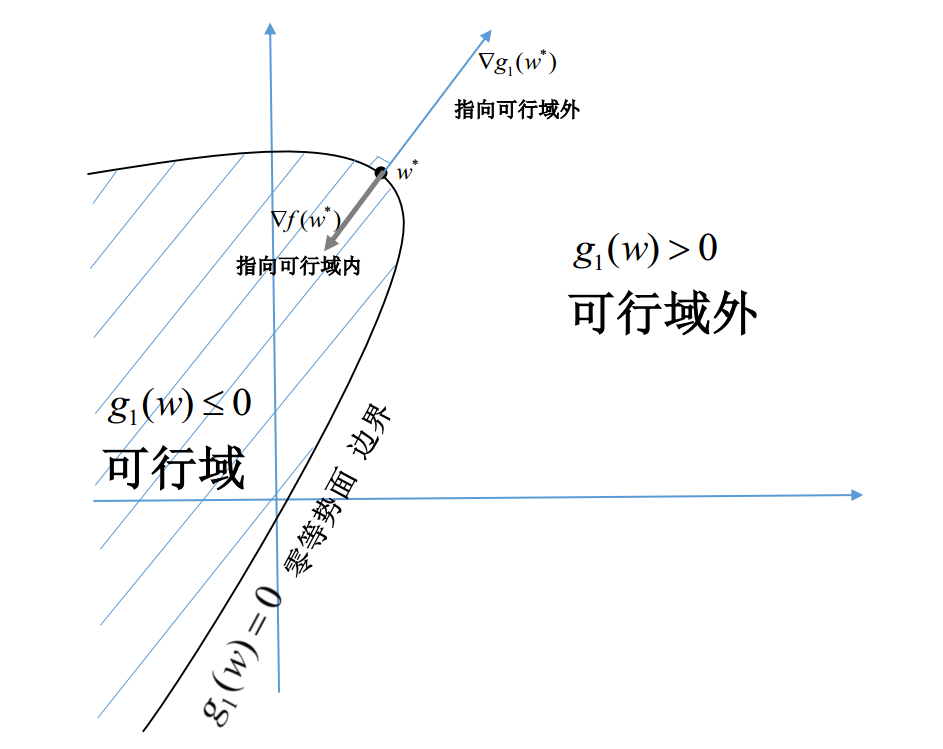现在可以做个小结了：若

w

∗

$w^*$是可行域上的极值点，则应满足：

{

∇

f

(

w

∗

)

+

α

1

∇

g

1

(

w

∗

)

=

0

且

α

1

≥

0

w

∗

是

边

界

点

，

即

g

1

(

w

∗

)

=

0

时

∇

f

(

w

∗

)

=

0

w

∗

是

内

点

，

即

g

1

(

w

∗

)

<

0

时

\begin{cases}
\nabla f(w^*)+\alpha_1\nabla g_1(w^*)=0且\alpha_1\ge0&w^*是边界点，即g_1(w^*)=0时\\
\nabla f(w^*)=0&w^*是内点，即g_1(w^*)<0时
\end{cases}
或者换一种更统一的形式

∇

f

(

w

∗

)

+

α

1

∇

g

1

(

w

∗

)

=

0

其

中

α

1

≥

0

，

且

当

g

1

(

w

∗

)

<

0

时

只

能

取

等

号

\nabla f(w^*)+\alpha_1\nabla g_1(w^*)=0\\
其中\alpha_1\ge0，且当g_1(w^*)<0时只能取等号

我们甚至可以把原问题中的约束条件也包进来，构造一个完整的方程组：

求

解

w

和

α

1

{

∇

f

(

w

)

+

α

1

∇

g

1

(

w

)

=

0

α

1

g

1

(

w

)

=

0

α

1

≥

0

,

g

1

(

w

)

≤

0

求解w和\alpha_1\begin{cases}
\nabla f(w)+\alpha_1\nabla g_1(w)=0\\
\alpha_1g_1(w)=0\\
\alpha_1\ge0,\ g_1(w)\le0
\end{cases}

巧妙的是，第二行的

(

α

1

g

1

(

w

)

=

0

)

$(\alpha_1g_1(w)=0)$和第三行的

(

α

1

≥

0

,

g

1

(

w

)

≤

0

)

$(\alpha_1\ge0,\ g_1(w)\le0)$联合起来，正好暗示了一个规则：当

g

1

(

w

)

<

0

$g_1(w)\lt0$时，

α

1

=

0

$\alpha_1=0$；只有当

g

1

(

w

)

=

0

$g_1(w)=0$时，

α

1

$\alpha_1$才可能大于0

同时存在多个不等式约束的情况

o

b

j

e

c

t

i

v

e

:

m

i

n

w

f

(

w

)

s

.

t

:

g

i

(

w

)

≤

0

,

i

=

1

,

2

,

3...

n

;

多个不等式约束同时存在时，思路与前面大致相同。
如果

w

∗

$w^*$不在边界上，只需判断

∇

f

(

w

∗

)

$\nabla f(w^*)$是否为0；
如果

w

∗

$w^*$只在其中某一个约束比如

g

i

(

w

)

≤

0

$g_i(w)\le0$的边界上，处理方法与前面一样；
唯一需要多加考虑的，是当

w

∗

$w^*$同时在多个约束的公共边界上的情况，即

w

∗

$w^*$在多个不同约束的边界面的交集上，比如，

g

1

(

w

∗

)

=

g

2

(

w

∗

)

=

g

3

(

w

∗

)

=

g

4

(

w

∗

)

=

0

$g_1(w^*)=g_2(w^*)=g_3(w^*)=g_4(w^*)=0$。下面以此为例来说明。
首先，为使

w

∗

$w^*$在公共边界上满足极值条件，

∇

f

(

w

∗

)

$\nabla f(w^*)$应与公共边界垂直。
而公共边界同时属于

g

1

(

w

∗

)

、

g

2

(

w

∗

)

、

g

3

(

w

∗

)

、

g

4

(

w

∗

)

$g_1(w^*)、g_2(w^*)、g_3(w^*)、g_4(w^*)$的0等势面，因此

∇

g

1

(

w

∗

)

、

∇

g

2

(

w

∗

)

、

∇

g

3

(

w

∗

)

、

∇

g

3

(

w

∗

)

$\nabla g_1(w^*)、\nabla g_2(w^*)、\nabla g_3(w^*)、\nabla g_3(w^*)$分别都与公共边界垂直，且这四个向量张成的四维空间正好构成公共边界的补空间，所有与公共边界垂直的向量，都在这个补空间中，可以被这四个向量的线性组合表示。
因此

∇

f

(

w

∗

)

+

∑

i

=

1

4

α

i

∇

g

i

(

w

∗

)

=

0

\nabla f(w^*)+\sum_{i=1}^{4}{\alpha_i\nabla g_i(w^*)}=0
同样，我们还要关心可行域上、公共边界以外的其他点。
设

(

w

∗

+

Δ

w

)

$(w^*+\Delta w)$是邻域内任一点，当

Δ

w

$\Delta w$同时满足如下条件时：

∇

g

i

(

w

∗

)

⋅

Δ

w

≤

0

，

其

中

i

=

1

,

2

,

3

,

4

\nabla g_i(w^*)\cdot\Delta w\le0，其中i=1,2,3,4

(

w

∗

+

Δ

w

)

$(w^*+\Delta w)$就是可行域上的点。
为使

f

(

w

)

$f(w)$ 能在

w

∗

$w^*$处取可行域上的极小值，对于可行域内的所有

Δ

w

$\Delta w$ 须满足

∇

f

(

w

∗

)

⋅

Δ

w

≥

0

$\nabla f(w^*)\cdot\Delta w\ge0$ ，即

−

∑

i

=

1

4

α

i

∇

g

i

(

w

∗

)

⋅

Δ

w

≥

0

-\sum_{i=1}^{4}{\alpha_i\nabla g_i(w^*)\cdot\Delta w}\ge0

同样的几何意义：

∇

f

(

w

∗

)

$\nabla f(w^*)$应与公共边界面垂直并指向靠近可行域的方向。  这里说的“靠近可行域的方向”，是指与可行域夹角小于等于90°的方向。准确的说，对于所有能使

(

w

∗

+

Δ

w

)

$(w^*+\Delta w)$位于可行域上的微小位移向量

Δ

w

$\Delta w$，与

∇

f

(

w

∗

)

$\nabla f(w^*)$夹角都应小于等于90°，用内积表示就是

∇

f

(

w

∗

)

⋅

Δ

w

≥

0

$\nabla f(w^*)\cdot\Delta w\ge0$

结合

∇

g

i

(

w

∗

)

⋅

Δ

w

≤

0

$\nabla g_i(w^*)\cdot\Delta w\le0$，为保证对所有满足的条件的

Δ

w

$\Delta w$上式都能成立，必须要求每个

α

i

$\alpha_i$都大于等于0。

否则，假如

α

1

$\alpha_1$小于0，对于满足以下条件

Δ

w

$\Delta w$，

∇

g

1

(

w

∗

)

⋅

Δ

w

<

0

,

∇

g

2

(

w

∗

)

⋅

Δ

w

=

0

,

∇

g

3

(

w

∗

)

⋅

Δ

w

=

0

,

∇

g

4

(

w

∗

)

⋅

Δ

w

=

0

\nabla g_1(w^*)\cdot\Delta w<0,\
\nabla g_2(w^*)\cdot\Delta w=0,\\
\nabla g_3(w^*)\cdot\Delta w=0,\
\nabla g_4(w^*)\cdot\Delta w=0上面的式子就不成立了

总结起来就是，当

w

∗

$w^*$在上述公共边界并是可行极值点时，应满足：

∇

f

(

w

∗

)

+

∑

i

=

1

n

α

1

∇

g

1

(

w

∗

)

=

0

仅

当

i

=

1

,

2

,

3

,

4

时

，

α

i

≥

0

，

其

他

α

i

=

0

\nabla f(w^*)+\sum_{i=1}^{n}{\alpha_1\nabla g_1(w^*)}=0\\
仅当i=1,2,3,4时，\alpha_i\ge0，其他\alpha_i=0

OK，有了这个特例的辅助，我们很容易将结论一般化如下：

∇

f

(

w

∗

)

+

∑

i

=

1

n

α

1

∇

g

1

(

w

∗

)

=

0

其

中

α

i

≥

0

，

且

当

g

i

(

w

∗

)

<

0

，

即

w

∗

不

在

第

i

个

约

束

边

界

时

必

须

取

等

号

\nabla f(w^*)+\sum_{i=1}^{n}\alpha_1\nabla g_1(w^*)=0\\
其中\alpha_i\ge0，且当g_i(w^*)<0，即w^*不在第i个约束边界时必须取等号

我们继续运用前面说到的巧妙办法，把原问题中的约束条件也包进来，构造一个完整的方程组：

求

解

w

和

α

{

∇

f

(

w

)

+

∑

i

=

1

n

α

i

∇

g

i

(

w

)

=

0

α

i

g

i

(

w

)

=

0

f

o

r

i

=

1

,

2...

n

α

i

≥

0

,

g

i

(

w

)

≤

0

f

o

r

i

=

1

,

2...

n

求解w和\alpha\begin{cases}
\nabla f(w)+ \sum_{i=1}^{n} {\alpha_i\nabla g_i(w)}=0\\
\alpha_ig_i(w)=0& for\ i=1,2...n\\
\alpha_i\ge0,\ g_i(w)\le0& for\ i=1,2...n
\end{cases}
现在到再请出拉格朗日大人的时候了。我们构造一个能把约束和目标函数融合在一起的新函数，如下（拉格朗日乘子式）：

L

(

w

,

α

)

=

f

(

w

)

+

∑

i

=

1

n

α

i

g

i

(

w

)

L(w,\alpha)=f(w)+\sum_{i=1}^{n}{\alpha_ig_i(w)}
这时上面的方程组变成了

求

解

w

和

α

{

∂

L

∂

w

k

=

0

f

o

r

k

=

1

,

2

,

3...

d

w

(

w

的

维

数

)

α

i

⋅

∂

L

∂

α

i

=

0

f

o

r

i

=

1

,

2...

n

α

i

≥

0

,

∂

L

∂

α

i

≤

0

f

o

r

i

=

1

,

2...

n

求解w和\alpha\begin{cases}
\frac{\partial L}{\partial w_k}=0&for\ k=1,2,3...d_w(w的维数)\\
\alpha_i\cdot\frac{\partial L}{\partial \alpha_i}=0&for\ i=1,2...n\\
\alpha_i\ge0,\frac{\partial L}{\partial \alpha_i}\le0&for\ i=1,2...n
\end{cases}
这里共有

d

w

+

n

$d_w+n$个等式方程和

d

w

+

n

$d_w+n$个变量，可以先求解前两行构成的等式方程组，再排除掉其中不符合第三行条件的解，剩下的即是符合条件候选极值点。
然而，第二行的方程与纯等式约束时的情况不太一样，纯等式约束下只要求约束式的偏导为0，而这里是要求偏导与系数(也是待求的未知数)乘积为0，这增加了解方程的难度。即便当目标函数是二次的、不等式约束都是线性的时候，方程组整体也变成了二次方程组，很难直接求解。不过，这时不等式约束的数量n是可以大于d_w的，因为第二行的方程不在只包含

w

$w$，同时也包含了$\alpha$$\alpha$，不会出现

w

$w$的约束太强而无解。

3. 混合约束时的optimalize问题——KKT条件与拉格朗日对偶问题

这一类问题的范式如下：
s.t.\
\begin{cases}
g_i(w)\le0, &i=1,2,3...n;&\text{不等式约束}\\
h_j(w)=0,&j=1,2,3...m;&\text{等式约束}
\end{cases}

g

i

(

w

)

$g_i(w)$,

h

j

(

w

)

$h_j(w)$和

f

(

w

)

$f(w)$都应是连续可导的。

拉格朗日乘子式与KKT条件
有了前面纯等式和纯不等式约束问题的铺垫，我们可以轻松得出混合约束时的结论。  首先，还是先考虑最简单的情况，假如

w

∗

$w^*$不在任何不等式的边界，即

g

i

(

w

∗

)

<

0

$g_i(w^*)<0$对所有

i

=

1

,

2...

n

$i=1,2...n$ 成立。——被玩坏的

w

∗

$w^*$肯定在抱怨：为什么又是我躺枪，隔壁

w

′

$w'$闲了两天了！但显然无情的笔者才不打算关心这个声音。  但

w

∗

$w^*$须仍在所有等式约束

h

j

(

w

)

$h_j(w)$的公共0势面上。这时相当于只有等式约束，

w

∗

$w^*$是极值的条件是：

∇

f

(

w

∗

)

+

∑

j

=

1

m

β

j

∇

h

j

(

w

∗

)

=

0

\nabla f(w^*)+\sum_{j=1}^{m}{\beta_j\nabla h_j(w^*)}=0

我们再把

w

∗

$w^*$扔到不等式的边界上看看会发生什么。有了之前纯不等式约束的基础，我们直接考虑

w

∗

$w^*$同时在多个不等式约束边界时的情况，假如

w

∗

$w^*$在第1、2、3、4个不等式约束的公共边界面上，即：

{

g

i

(

w

∗

)

=

0

f

o

r

i

=

1

,

2

,

3

,

4

g

i

(

w

∗

)

<

0

f

o

r

i

=

o

t

h

e

r

h

j

(

w

∗

)

=

0

f

o

r

j

=

1

,

2...

m

\begin{cases}
g_i(w^*)=0&for\ i=1,2,3,4\\
g_i(w^*)<0&for\ i=other\\
h_j(w^*)=0&for\ j=1,2...m
\end{cases}
可见，这个公共边界面，属于

g

1

(

w

)

、

g

2

(

w

)

、

g

3

(

w

)

、

g

4

(

w

)

$g_1(w)、g_2(w)、g_3(w)、g_4(w)$和

h

1

(

w

)

、

h

2

(

w

)

.

.

.

h

m

(

w

)

$h_1(w)、h_2(w)...h_m(w)$的公共混合0势面的一部分。如果想让

f

(

w

)

$f(w)$在

w

∗

$w^*$处是可行域上的一个极值，首先要保证

w

∗

$w^*$在这个公共混合0势面上是极值，即

f

(

w

)

$f(w)$在

w

∗

$w^*$处的梯度应垂直于该混合0势面，可以表示成：

∇

f

(

w

∗

)

+

∑

j

=

1

m

β

j

∇

h

j

(

w

∗

)

+

∑

i

=

1

4

α

i

∇

g

i

(

w

∗

)

=

0

\nabla f(w^*)+\sum_{j=1}^{m}{\beta_j\nabla h_j(w^*)}+\sum_{i=1}^{4}{\alpha_i\nabla g_i(w^*)}=0

但这还不够，为了保证可行域上

w

∗

$w^*$所有邻域点

(

w

∗

+

Δ

w

)

$(w^*+\Delta w)$的目标函数值都比

w

∗

$w^*$处大，

α

1

.

.

.

α

4

$\alpha_1...\alpha_4$还应都是大于等于0的，具体分析过程与讲解纯不等式约束问题时类似，不再重复。

再强调下几何意义：

∇

f

(

w

∗

)

$\nabla f(w^*)$应与公共混合0势面垂直并指向靠近可行域的方向。

将上面两种情况用统一的形式重写如下，

w

∗

$w^*$是可行域上极值点的条件是：

∇

f

(

w

∗

)

+

∑

j

=

1

m

β

j

∇

h

j

(

w

∗

)

+

∑

i

=

1

n

α

1

∇

g

1

(

w

∗

)

=

0

其

中

α

i

≥

0

，

且

当

g

i

(

w

∗

)

<

0

，

即

w

∗

不

在

第

i

个

不

等

式

约

束

边

界

时

只

能

取

等

号

\nabla f(w^*)+\sum_{j=1}^{m}{\beta_j\nabla h_j(w^*)}+\sum_{i=1}^{n}\alpha_1\nabla g_1(w^*)=0\\
其中\alpha_i\ge0，且当g_i(w^*)<0，即w^*不在第i个不等式约束边界时只能取等号

因此，我们可以通过解以下方程组（前3行等式构成方程组）

求

解

w

、

α

和

β

{

∇

f

(

w

)

+

∑

j

=

1

m

β

j

∇

h

j

(

w

)

+

∑

i

=

1

n

α

i

∇

g

i

(

w

)

=

0

h

j

(

w

)

=

0

f

o

r

j

=

1

,

2...

m

α

i

g

i

(

w

)

=

0

f

o

r

i

=

1

,

2...

n

α

i

≥

0

,

g

i

(

w

)

≤

0

f

o

r

i

=

1

,

2...

n

求解w、\alpha和\beta\begin{cases}
\nabla f(w)+ \sum_{j=1}^{m} {\beta_j\nabla h_j(w)}+\sum_{i=1}^{n} {\alpha_i\nabla g_i(w)}=0\\
h_j(w)=0& for\ j=1,2...m\\
\alpha_ig_i(w)=0& for\ i=1,2...n\\
\alpha_i\ge0,g_i(w)\le0& for\ i=1,2...n
\end{cases}
然后排除掉不满足第4行的解，就可以得到可行域上的候选极值点。

然后，拉格朗日时间到。是时候引出完整版的拉格朗日乘子式了，我们构造一个融合目标函数和所有约束的新函数：

L

(

w

,

α

,

β

)

=

f

(

w

)

+

∑

i

=

1

n

α

i

g

i

(

w

)

+

∑

j

=

1

m

β

j

h

j

(

w

)

L(w,\alpha,\beta)=f(w)+\sum_{i=1}^n{\alpha_ig_i(w)}+\sum_{j=1}^m{\beta_jh_j(w)} \\

然后前面的方程组就变成了

求

解

w

、

α

和

β

{

∂

L

∂

w

k

=

0

f

o

r

k

=

1

,

2

,

3...

d

w

(

w

的

维

数

)

∂

L

∂

β

j

=

0

f

o

r

j

=

1

,

2...

m

α

i

⋅

∂

L

∂

α

i

=

0

f

o

r

i

=

1

,

2...

n

α

i

≥

0

,

∂

L

∂

α

i

≤

0

f

o

r

i

=

1

,

2...

n

求解w、\alpha和\beta\begin{cases}
\frac{\partial L}{\partial w_k}=0&for\ k=1,2,3...d_w(w的维数)\\
\frac{\partial L}{\partial \beta_j}=0&for\ j=1,2...m\\
\alpha_i\cdot \frac{\partial L}{\partial \alpha_i}=0&for\ i=1,2...n\\
\alpha_i\ge0,\frac{\partial L}{\partial \alpha_i}\le0& for\ i=1,2...n
\end{cases}

是的，我们就这样得到了广为流传的KKT条件。对于某组给定的

(

w

∗

,

α

∗

,

β

∗

)

$(w^*,\alpha^*,\beta^*)$，如果能同时满足上面的条件，

w

∗

$w^*$就是原问题的候选解，但候选解不一定是最优解。KKT条件只是最优解的必要条件。
拉格朗日对偶问题
前面KKT条件中的方程组通常是很难直接求解的。而有些时候，将原问题转换成另一种形式，可能更会容易求解。下面就介绍一种常见的转换形式，对偶问题。
我们先来看一个与原问题等价的问题。设

θ

(

w

)

=

max

α

≥

0

,

β

L

(

w

,

α

,

β

)

注意条件，所有的

α

i

≥

0

注意所有的

α

i

$\alpha_i$ 都是大于等于0的。  容易得出

θ

(

w

)

=

{

f

(

w

)

所有等式和不等式约束均被满足

+

∞

,

有任意一个约束未被满足

\theta(w)=\begin{cases}
f(w)& \text{所有等式和不等式约束均被满足}\\
+\infty,&\text{有任意一个约束未被满足}
\end{cases}

如果不等式约束中有任意一个约束未被满足，比如若某个

w

∗

$w^*$ 使得

g

i

(

w

∗

)

>

0

$g_i(w^*)>0$ 时, 那我们将对应的

α

i

$\alpha_i$ 取

+

∞

$+\infty$ ，则

α

i

g

i

(

w

∗

)

$\alpha_ig_i(w^*)$ 必然也是

+

∞

$+\infty$ ，导致

θ

(

w

∗

)

$\theta(w^*)$ 变为

+

∞

$+\infty$ 。  同理如果原问题的等式约束中有任意一个约束未被满足，比如若某个

w

∗

$w^*$使得

h

j

(

w

∗

)

≠

0

$h_j(w^*)\ne0$时, 那我们将对应的

β

j

$\beta_j$ 取

β

j

=

{

−

∞

h

j

(

w

∗

)

<

0

+

∞

,

h

j

(

w

∗

)

>

0

\beta_j=\begin{cases}
-\infty& h_j(w^*)\lt0\\
+\infty,& h_j(w^*)\gt0
\end{cases}
则

β

j

h

j

(

w

∗

)

$\beta_jh_j(w^*)$必然是

+

∞

$+\infty$，，导致

θ

(

w

∗

)

$\theta(w^*)$ 变为

+

∞

$+\infty$。
只有当

w

∗

$w^*$满足所有的等式约束和不等式约束时，

θ

(

w

∗

)

$\theta(w^*)$才不会变为无穷大。而且由于此时等式约束被满足，导致所有的

β

j

h

j

(

w

∗

)

$\beta_jh_j(w^*)$项被直接消去，因为他们都等于0；另一方面由于所有的

α

i

≥

0

,

g

i

(

w

∗

)

≤

0

$\alpha_i\ge0, g_i(w^*)\le0$ ，因此

α

i

g

i

(

w

∗

)

$\alpha_ig_i(w^*)$ 取最大时必然也是0，即所有的

α

i

g

i

(

w

∗

)

$\alpha_ig_i(w^*)$项也被消去，只剩下了

f

(

w

)

$f(w)$。于是就有上面的结果

θ

(

w

)

=

{

f

(

w

)

constraint satisfied

+

∞

,

constraint not satisfied

\theta(w)=\begin{cases}
f(w)& \text{constraint satisfied}\\
+\infty,&\text{constraint not satisfied}
\end{cases}

这样一来，求原问题中的

min

w

f

(

w

)

$\min_w f(w)$ 就等价于求

min

w

θ

(

w

)

$\min_w \theta(w)$ 。  原问题等价于：

o

b

j

e

c

t

i

v

e

:

min

w

max

α

,

β

L

(

w

,

α

,

β

)

s

.

t

.

α

i

≥

0

,

for i=1,2,3...n

如果调换上面 min 和 max 的顺序，就得到该问题的对偶问题。  对偶问题 ：

o

b

j

e

c

t

i

v

e

:

max

α

,

β

min

w

L

(

w

,

α

,

β

)

s

.

t

.

α

i

≥

0

,

for i=1,2,3...n

引入一个新函数：

θ

D

(

α

,

β

)

=

min

w

L

(

w

,

α

,

β

)

$\theta_D(\alpha,\beta)=\min_{w} L(w,\alpha,\beta)$ ，这时对偶问题变成：

o

b

j

e

c

t

i

v

e

:

max

α

,

β

θ

D

(

α

,

β

)

s

.

t

.

α

i

≥

0

,

for i=1,2,3...n

根据

θ

D

$\theta_D$的定义可知，对于任意的

(

w

,

β

,

α

≥

0

)

$(w,\beta,\alpha\ge0)$，有

θ

D

(

α

,

β

)

≤

L

(

w

,

α

,

β

)

$\theta_D(\alpha,\beta)\le L(w,\alpha,\beta)$；  根据

θ

$\theta$的定义可知，对于任意的

(

w

,

β

,

α

≥

0

)

$(w,\beta,\alpha\ge0)$，有

θ

(

w

)

≥

L

(

w

,

α

,

β

)

$\theta(w)\ge L(w,\alpha,\beta)$；  如果设

p

∗

=

θ

(

w

∗

)

=

min

w

θ

(

w

)

,

d

∗

=

θ

D

(

α

∗

,

β

∗

)

=

max

α

≥

0

,

β

θ

D

(

α

,

β

)

p^*=\theta(w^*)=\min_w\theta(w),\\
d^*= \theta_D(\alpha^*,\beta^*)=\max_{\alpha\ge0,\beta}\theta_D(\alpha,\beta)
则

d

∗

=

θ

D

(

α

∗

,

β

∗

)

≤

L

(

w

∗

,

α

∗

,

β

∗

)

≤

θ

(

w

∗

)

=

p

∗

d^*= \theta_D(\alpha^*,\beta^*)\le L(w^*,\alpha^*,\beta^*)\le \theta(w^*)=p^*
即

d

∗

≤

p

∗

$d^*\le p^*$，这个特性被称为”弱对偶性”，

(

p

∗

−

d

∗

)

$(p^*-d^*)$被称为”对偶间隙”，对偶间隙是大于等于0的。通过求解对偶问题，可以求出原问题的一个下限，在实际中这对寻求原问题最优解有一定的帮助。
如果对偶间隙为0即

d

∗

=

p

∗

$d^*= p^*$，则称之为”强对偶性”，此时对偶问题于原问题等价，只需求解对偶问题即可，

(

w

∗

,

α

∗

,

β

∗

)

$(w^*,\alpha^*,\beta^*)$同时是原问题和对偶问题的解。
那什么情况下强对偶性才能被满足呢？我们介绍一个能在
凸优化问题中保证强对偶性的充分条件，下一节再证明这个结论。

定义: 凸优化问题  当且仅当目标函数

f

(

w

)

$f(w)$和所有的不等式约束函数

g

i

(

w

)

$g_i(w)$都是凸函数，所有的等式约束

h

j

(

w

)

$h_j(w)$都是线性(仿射)函数时，相应的最优化问题属于凸优化问题。  Slater条件  对于凸优化问题，如果可行域满足Slater条件，强对偶性保证成立。  Slater条件是指，可行域存在一点

w

′

$w'$同时满足：

{

g

i

(

w

′

)

<

0

f

o

r

i

=

1

,

2...

n

(

注

意

是

<

不

再

是

≤

)

h

j

(

w

′

)

=

0

f

o

r

j

=

1

,

2...

m

\begin{cases}
g_i(w')<0&for\ i=1,2...n\ (注意是<不再是\le)\\
h_j(w')=0&for\ j=1,2...m
\end{cases}
如果不等式约束中存在线性约束，比如前

t

$t$个不等式约束都是线性约束时，Slater条件可以弱化为：\begin{cases}
g_i(w')\le0&for\ i=1,2...t\\
g_i(w')<0&for\ i=t+1,t+2...n\\
h_j(w')=0&for\ j=1,2...m
\end{cases}

KKT条件的充分性
之前的分析中，KKT条件只能作为最优解的必要条件，但满足KKT条件的解不一定是最优解。而对于凸优化问题，KKT条件同时也是最优解的充分条件。

如果

(

w

∗

,

α

∗

,

β

∗

)

$(w^*,\alpha^*,\beta^*)$是满足KKT条件的一组解，即

{

∂

L

∂

w

k

∗

=

0

f

o

r

k

=

1

,

2

,

3...

d

w

(

w

的

维

数

)

∂

L

∂

β

j

∗

=

0

f

o

r

j

=

1

,

2...

m

α

i

∗

⋅

∂

L

∂

α

i

∗

=

0

f

o

r

i

=

1

,

2...

n

α

i

∗

≥

0

,

∂

L

∂

α

i

∗

≤

0

f

o

r

i

=

1

,

2...

n

\begin{cases}
\frac{\partial L}{\partial w^*_k}=0&for\ k=1,2,3...d_w(w的维数)\\
\frac{\partial L}{\partial \beta^*_j}=0&for\ j=1,2...m\\
\alpha^*_i\cdot \frac{\partial L}{\partial \alpha^*_i}=0&for\ i=1,2...n\\
\alpha^*_i\ge0,\frac{\partial L}{\partial \alpha^*_i}\le0& for\ i=1,2...n
\end{cases}
由于

α

∗

≥

0

$\alpha^*\ge0$，以及凸优化问题中各不等式约束函数和目标函数都是凸函数的特点，易知

L

(

w

,

α

∗

,

β

∗

)

$L(w,\alpha^*,\beta^*)$是

w

$w$的凸函数，而KKT条件的第一行说明${w}^{\ast }$$w^*$是使得

L

(

w

,

α

∗

,

β

∗

)

$L(w,\alpha^*,\beta^*)$对

w

$w$导数为0的点，因此
$L\left({w}^{\ast },{\alpha }^{\ast },{\beta }^{\ast }\right)=\underset{w}{min}L\left(w,{\alpha }^{\ast },{\beta }^{\ast }\right)={\theta }_{D}\left({\alpha }^{\ast },{\beta }^{\ast }\right)$L(w^*,\alpha^*,\beta^*)=\min_w{L(w,\alpha^*,\beta^*)}=\theta_D(\alpha^*,\beta^*)
同时，KKT条件的第二行

h

(

w

∗

)

=

0

$h(w^*)=0$和第三行

α

∗

g

(

w

∗

)

=

0

$\alpha^*g(w^*)=0$使得

L

(

w

∗

,

α

∗

,

β

∗

)

=

f

(

w

∗

)

=

θ

(

w

∗

)

L(w^*,\alpha^*,\beta^*)=f(w^*)=\theta(w^*)
因此有

θ

D

(

α

∗

,

β

∗

)

=

θ

(

w

∗

)

$\theta_D(\alpha^*,\beta^*)=\theta(w^*)$。
而同时

θ

D

(

α

∗

,

β

∗

)

≤

d

∗

≤

p

∗

≤

θ

(

w

∗

)

$\theta_D(\alpha^*,\beta^*)\le d^*\le p^*\le \theta(w^*)$ ，这其实暗示了

d

∗

=

p

∗

$d^*= p^*$ ，即强对偶性成立，且

(

w

∗

,

α

∗

,

β

∗

)

$(w^*,\alpha^*,\beta^*)$ 正好是原问题和对偶问题的最优解。
因此，对于凸优化问题，满足KKT条件的解一定是最优解，且满足KKT条件暗示了强对偶性成立。

4. 强对偶性的证明与Slater条件
强对偶性的一种比较直观的证明是用几何方法。
先介绍一条关于凸集的定理。这个定理表达的意思很直观。比如在二维空间中，二维平面上的任意一个凸图形（比如一个圆），在其边缘上任一点处做切线，该图形必在切线的同一侧，而不会被切割成多个部分并分居两侧。该定理的完整表述如下：

引理： 支撑超平面定理  设集合

C

$C$ 是 ${\mathbb{R}}^{n}$$\mathbb{R}^n$ 空间中的闭凸集，

x

¯

$\bar{\boldsymbol{x}}$ 是

C

$C$ 边界上一点，则必存在一个过点 $\overline{\mathbit{x}}$$\bar{\boldsymbol{x}}$ 的超平面，使得

C

$C$ 位于它的一个闭半空间。即存在法向量 $\mathbit{a}$$\boldsymbol{a}$ ，使得对于

C

$C$ 中任意点 $\mathbit{x}$$\boldsymbol{x}$ ，有：

a

⋅

x

−

a

⋅

x

¯

≥

0

\boldsymbol{a}\cdot\boldsymbol{x}-\boldsymbol{a}\cdot\bar{\boldsymbol{x}}\ge0
进一步地，如果已知

x

i

n

$\boldsymbol{x}^{in}$ 是

C

$C$ 的一个内点，则：
$\mathbit{a}\cdot {\mathbit{x}}^{in}-\mathbit{a}\cdot \overline{\mathbit{x}}>0$\boldsymbol{a}\cdot\boldsymbol{x}^{in}-\boldsymbol{a}\cdot\bar{\boldsymbol{x}}\gt0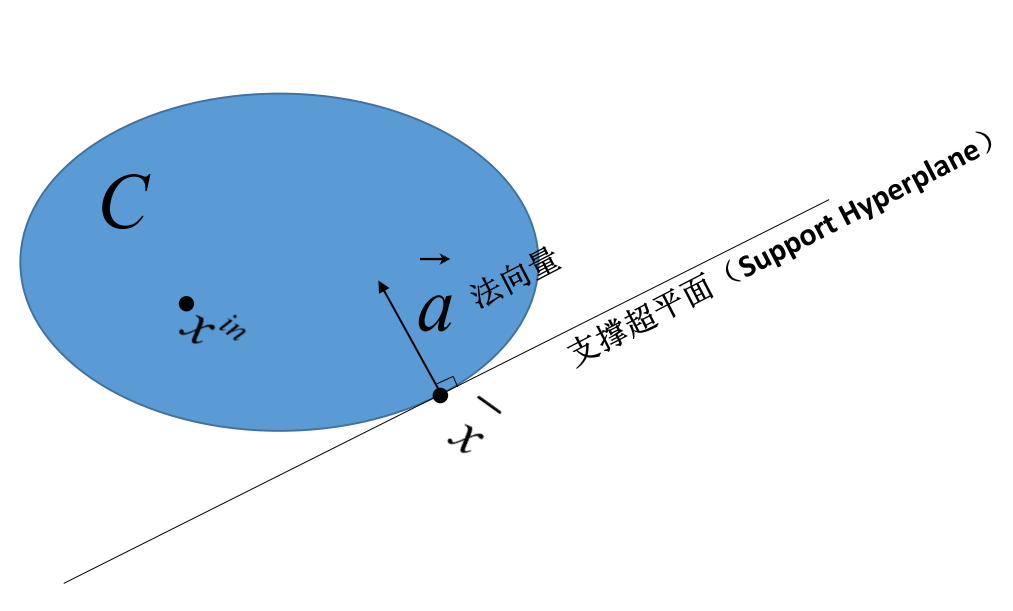如果想了解该定理的证明看这里：

http://www.cnblogs.com/murongxixi/p/3615034.html
这是在网上找到的一篇博文，里面步骤比较详尽。为防止原链接失效，相关的重要证明步骤会以图片形式插入到本文末尾的附录中。

回到我们的问题。我们要研究的是凸优化问题中强对偶性成立的条件，首先做一个基本假设：

基本假设：假设原问题是凸优化问题，即

f

(

w

)

,

g

i

(

w

)

$f(w),g_i(w)$都是凸函数，

h

j

(

w

)

$h_j(w)$都是线性函数，且所有的

h

j

(

w

)

$h_j(w)$相互之间都是线性独立的。

w

∈

R

d

w

$w\in \mathbb{R}^{d_w}$，我们按如下方式将

R

d

w

$\mathbb{R}^{d_w}$空间中一点

w

$w$映射为${\mathbb{R}}^{\left(n+m+1\right)}$$\mathbb{R}^{(n+m+1)}$空间中的点

E

$E$：
$E\left(w\right)=\left({u}_{1},{u}_{2},...{u}_{n},{v}_{1},{v}_{2},...{v}_{m},t\right)\phantom{\rule{0ex}{0ex}}其中\left\{\begin{array}{ll}{u}_{i}={g}_{i}\left(w\right)& i=1,2,3...n\\ {v}_{j}={h}_{j}\left(w\right)& j=1,2,3...m\\ t=f\left(w\right)\end{array}$E(w)=(u_1,u_2,...u_n,v_1,v_2,...v_m,t)\\
其中\begin{cases}
u_i=g_i(w)&i=1,2,3...n\\
v_j=h_j(w)&j=1,2,3...m\\
t=f(w)\\
\end{cases}  简化表示为

E

=

(

u

,

v

,

t

)

$E=(u,v,t)$。
设所有点

E

$E$组成的集合为${G}_{o}$$G_o$，

G

o

=

{

(

u

,

v

,

t

)

:

u

=

g

(

w

)

,

v

=

h

(

w

)

,

t

=

f

(

w

)

,

w

∈

R

d

w

}

$G_o=\{(u,v,t):u=g(w),v=h(w),t=f(w),w\in \mathbb{R}^{d_w}\}$  然而，即便有了前面的基本假设，我们也不能保证

G

o

$G_o$是凸集。但是如果把它简单扩展一下，设：

G

e

=

{

(

u

,

v

,

t

)

:

u

≥

g

(

w

)

,

v

=

h

(

w

)

,

t

≥

f

(

w

)

,

w

∈

R

d

w

}

G_e=\{(u,v,t):u\ge g(w),v=h(w),t\ge f(w),w\in \mathbb{R}^{d_w}\}

容易证明

G

e

$G_e$是一个凸集，且

G

o

⊆

G

e

$G_o \subseteq G_e$。

若

A

(

u

a

,

v

a

,

t

a

)

$A(u_a,v_a,t_a)$和

B

(

u

b

,

v

b

,

t

b

)

$B(u_b,v_b,t_b)$是

G

e

$G_e$内的两个点，按照

G

e

$G_e$的定义可知必然存在

w

a

$w_a$和

w

b

$w_b$，使得

v

a

=

h

(

w

a

)

,

u

a

≥

g

(

w

a

)

,

t

a

≥

f

(

w

a

)

v

b

=

h

(

w

b

)

,

u

b

≥

g

(

w

b

)

,

t

b

≥

f

(

w

b

)

v_a=h(w_a),u_a\ge g(w_a),t_a\ge f(w_a)\\v_b=h(w_b),u_b\ge g(w_b),t_b\ge f(w_b)
设

0

≤

c

≤

1

,

w

c

=

c

w

a

+

(

1

−

c

)

w

b

,

C

=

c

A

+

(

1

−

c

)

B

=

(

u

c

,

v

c

,

t

c

)

$0\le c\le1, w_c=cw_a+(1-c)w_b,C=cA+(1-c)B=(u_c,v_c,t_c)$，则

u

c

=

c

u

a

+

(

1

−

c

)

u

b

v

c

=

c

v

a

+

(

1

−

c

)

v

b

t

c

=

c

t

a

+

(

1

−

c

)

t

b

u_c=cu_a+(1-c)u_b\\v_c=cv_a+(1-c)v_b\\t_c=ct_a+(1-c)t_b
由于

h

(

w

)

$h(w)$是

w

$w$的线性函数，因此
$c\cdot h\left({w}_{a}\right)+\left(1-c\right)h\left({w}_{b}\right)=h\left[c{w}_{a}+\left(1-c\right){w}_{b}\right]=h\left({w}_{c}\right)$c\cdot h(w_a)+(1-c)h(w_b)=h[cw_a+(1-c)w_b]=h(w_c)
即

v

c

=

h

(

w

c

)

$v_c = h(w_c)$；
由于

g

(

w

)

,

f

(

w

)

$g(w),f(w)$都是

w

$w$的凸函数，因此
$c\cdot g\left({w}_{a}\right)+\left(1-c\right)g\left({w}_{b}\right)\ge g\left[c{w}_{a}+\left(1-c\right){w}_{b}\right]=g\left({w}_{c}\right)\phantom{\rule{0ex}{0ex}}c\cdot f\left({w}_{a}\right)+\left(1-c\right)f\left({w}_{b}\right)\ge f\left[c{w}_{a}+\left(1-c\right){w}_{b}\right]=f\left({w}_{c}\right)$c\cdot g(w_a)+(1-c)g(w_b)\ge g[cw_a+(1-c)w_b]=g(w_c)\\
c\cdot f(w_a)+(1-c)f(w_b)\ge f[cw_a+(1-c)w_b]=f(w_c)
而同时

u

a

≥

g

(

w

a

)

,

t

a

≥

f

(

w

a

)

,

u

b

≥

g

(

w

b

)

,

t

b

≥

f

(

w

b

)

$u_a\ge g(w_a),t_a\ge f(w_a),u_b\ge g(w_b),t_b\ge f(w_b)$，于是

c

u

a

+

(

1

−

c

)

u

b

≥

c

⋅

g

(

w

a

)

+

(

1

−

c

)

g

(

w

b

)

c

t

a

+

(

1

−

c

)

t

b

≥

c

⋅

f

(

w

a

)

+

(

1

−

c

)

f

(

w

b

)

cu_a+(1-c)u_b\ge c\cdot g(w_a)+(1-c)g(w_b)\\
ct_a+(1-c)t_b\ge c\cdot f(w_a)+(1-c)f(w_b)
即

u

c

≥

g

(

w

c

)

,

t

c

≥

f

(

w

c

)

$u_c\ge g(w_c),t_c\ge f(w_c)$。再结合

v

c

=

h

(

w

c

)

$v_c = h(w_c)$，可知

(

u

c

,

v

c

,

t

c

)

∈

G

e

$(u_c,v_c,t_c)\in G_ e$，即

C

=

c

A

+

(

1

−

c

)

B

∈

G

e

$C=cA+(1-c)B\in G_ e$。由于前述

A

,

B

,

c

$A,B,c$ 的选取具有任意性，因此对于任意两点

A

,

B

∈

G

e

$A,B\in G_e$，连线

A

B

¯

$\overline{AB}$上的所有点都属于

G

e

$G_ e$，

G

e

$G_ e$是凸集。

除此之外，我们还知道

E

∗

=

(

0

,

0

,

p

∗

)

$E^*=(0,0,p^*)$ 必是

G

e

$G_ e$ 的一个边界点，其中

p

∗

=

min

w

θ

(

w

)

$p^*=\min_w\theta(w)$，

θ

(

w

)

$\theta(w)$的定义同上一节。

若

w

∗

$w^*$ 是目标函数

f

(

w

)

$f(w)$ 在可行域内的极小点，则

p

∗

=

f

(

w

∗

)

$p^*=f(w^*)$，且由于在可行域内，必然满足

g

(

w

∗

)

≤

0

$g(w^*)\le0$、

h

(

w

∗

)

=

0

$h(w^*)=0$，因此

(

0

,

0

,

p

∗

)

∈

G

e

$(0,0,p^*)\in G_e$;  又由于

p

∗

$p^*$ 是可行域上即区间

{

g

(

w

∗

)

≤

0

,

h

(

w

∗

)

=

0

}

$\{g(w^*)\le0,h(w^*)=0\}$上的最小值(边界值)，对于任意小的正数

δ

$\delta$，

(

0

,

0

,

p

∗

−

δ

)

∉

G

e

$(0,0,p^*-\delta)\not\in G_e$，因此

(

0

,

0

,

p

∗

)

$(0,0,p^*)$ 在边界上。  即

E

∗

=

(

0

,

0

,

p

∗

)

$E^*=(0,0,p^*)$ 是

G

e

$G_ e$ 的一个边界点。

利用上面关于边界点的支撑超平面定理，我们知道必存在一个法向量

a

=

(

α

a

,

β

a

,

γ

a

)

$\boldsymbol{a}=(\alpha_a,\beta_a,\gamma_a)$，使得对于

G

e

$G_e$内任一点

E

e

=

(

u

e

,

v

e

,

t

e

)

$E_e=(u_e,v_e,t_e)$，满足：

((1-a))

a

⋅

E

e

−

a

⋅

E

∗

≥

0

即

α

a

u

e

+

β

a

v

e

+

γ

a

t

e

≥

γ

a

p

∗

\boldsymbol{a}\cdot E_e - \boldsymbol{a}\cdot E^*\ge 0\\
即\quad \alpha_a u_e+\beta_a v_e +\gamma_a t_e\ge \gamma_a p^*\tag{(1-a)}

由于

u

e

,

t

e

$u_e,t_e$可以随意取正无穷大，

p

∗

$p^*$是个定值，为使上面的大于等于恒成立，

α

a

$\alpha_a$和

γ

a

$\gamma_a$必须大于等于0。否则，如果他们任意一个取负，当

u

e

,

t

e

$u_e,t_e$取正无穷大时，左边会变成负无穷而导致上式无法被满足。

h

(

w

)

$h(w)$是

w

$w$的线性函数，因此${v}_{e}=h\left(w\right)$$v_e=h(w)$也可以取到正无穷，甚至负无穷也可以。但

v

e

$v_e$不能单独、随意 地增大，因为

v

e

$v_e$完全由

w

$w$确定，而${u}_{e}和{t}_{e}$$u_e和t_e$只是被

w

$w$确定了下限，它们可以单独增大，且仍保证在集合${G}_{e}$$G_e$内。

γ

a

>

0

$\gamma_a\gt 0$的情况
假如

γ

a

≠

0

$\gamma_a\ne0$，此时令

α

∗

=

α

a

/

γ

a

,

β

∗

=

β

a

/

γ

a

$\alpha^* = \alpha_a / \gamma_a, \beta^* = \beta_a / \gamma_a$，则式(1-a)可重写如下：

((2-a))

α

∗

u

e

+

β

∗

v

e

+

t

e

≥

p

∗

\alpha^* u_e+\beta^* v_e+t_e\ge p^*\tag{(2-a)}
由于

α

a

≥

0

$\alpha_a\ge0$，

α

∗

≥

0

$\alpha^*\ge0$。
由于

G

o

⊆

G

e

$G_o \subseteq G_e$，因此

G

o

$G_o$中任意一点

E

(

u

,

v

,

t

)

=

(

g

(

w

)

,

h

(

w

)

,

f

(

w

)

)

$E(u,v,t)=(g(w), h(w), f(w))$也都满足上式。
即必然存在一组

α

∗

,

β

∗

$\alpha^*,\beta^*$，对任意的

w

∈

R

d

w

$w\in \mathbb{R}^{d_w}$，始终满足：

α

∗

g

(

w

)

+

β

∗

h

(

w

)

+

f

(

w

)

≥

p

∗

α

≥

0

\alpha^* g(w)+\beta^* h(w)+f(w)\ge p^*\\\alpha\ge0
我们上面都使用了简化后的表示方式，如果将上式完整展开，就是：存在一组

α

∗

,

β

∗

$\alpha^*,\beta^*$使得下式对所有的

w

∈

R

d

w

$w\in \mathbb{R}^{d_w}$成立。

∑

i

=

1

n

α

i

∗

g

i

(

w

)

+

∑

j

=

1

m

β

j

∗

h

j

(

w

)

+

f

(

w

)

=

L

(

w

,

α

∗

,

β

∗

)

≥

p

∗

α

i

∗

≥

0

,

f

o

r

i

=

1

,

2

,

3...

n

\sum_{i=1}^n\alpha^*_ig_i(w)+\sum_{j=1}^m\beta^*_jh_j(w)+f(w)=L(w,\alpha^*,\beta^*)\ge p^*\\
\alpha^*_i \ge 0, \quad for \ i=1,2,3...n

由于上式对任意的

w

$w$都成立，因此
${\theta }_{D}\left({\alpha }^{\ast },{\beta }^{\ast }\right)=\underset{w}{min}L\left(w,{\alpha }^{\ast },{\beta }^{\ast }\right)\ge {p}^{\ast }$\theta_D(\alpha^*,\beta^*)=\min_w L(w,\alpha^*,\beta^*)\ge p^*  进一步由于

d

∗

=

max

α

≥

0

,

β

θ

D

(

α

,

β

)

≥

θ

D

(

α

∗

,

β

∗

)

d^*=\max_{\alpha\ge0,\beta}{\theta_D(\alpha,\beta)}\ge\theta_D(\alpha^*,\beta^*)
于是得到

d

∗

≥

p

∗

$d^*\ge p^*$。而由弱对偶性，

d

∗

≤

p

∗

$d^*\le p^*$。因此

d

∗

=

p

∗

$d^*= p^*$，强对偶性成立。

γ

a

=

0

$\gamma_a=0$的情况，Slater条件的作用
这不是我们期望出现的情况，因为一旦

γ

a

=

0

$\gamma_a=0$，我们就无法将式(1-a)的左边转换成跟

L

(

w

,

α

,

β

)

$L(w,\alpha,\beta)$相同的形式了。实际上，只要上节中提到的Slater条件被满足（通常这个条件都是满足的），就不会出现

γ

a

=

0

$\gamma_a=0$的情况。下面我们分析为什么不会出现。
假如

γ

a

=

0

$\gamma_a=0$，此时式(1-a)变成

((4-a))

α

a

u

e

+

β

a

v

e

+

γ

a

t

e

≥

0

\alpha_a u_e+\beta_a v_e +\gamma_a t_e\ge 0\tag{(4-a)}

Slater条件保证了在可行域内，存在

w

′

$w'$ 使得

g

(

w

′

)

<

0

,

h

(

w

′

)

=

0

$g(w')<0,h(w')=0$，设

E

1

=

(

g

(

w

′

)

,

h

(

w

′

)

,

f

(

w

′

)

)

∈

G

e

E

2

=

(

g

(

w

′

)

+

Δ

g

,

h

(

w

′

)

,

f

(

w

′

)

+

Δ

f

)

∈

i

n

t

G

e

其

中

Δ

g

和

Δ

f

是

任

意

大

于

0

的

值

，

这

两

个

正

增

量

保

证

了

E

2

是

G

e

的

内

点

E_1=(g(w'),h(w'),f(w'))\in G_e\\
E_2=(g(w')+\Delta_g,h(w'),f(w')+\Delta_f)\in int\ G_e\\
其中\Delta_g和\Delta_f是任意大于0的值，这两个正增量保证了E_2是G_e的内点

则有

(4-b)

α

a

g

(

w

′

)

+

β

a

h

(

w

′

)

+

γ

a

f

(

w

′

)

≥

0

\alpha_a g(w')+\beta_a h(w') +\gamma_a f(w')\ge 0\tag{4-b}

(4-c)

α

a

[

g

(

w

′

)

+

Δ

g

]

+

β

a

h

(

w

′

)

+

γ

a

[

f

(

w

′

)

+

Δ

f

]

>

0

\alpha_a [g(w')+\Delta_g]+\beta_a h(w') +\gamma_a [f(w')+\Delta_f]\gt 0\tag{4-c}
式(4-c)中可以用大于号是因为

E

2

是

G

e

的

内

点

$E_2是G_e的内点$，上面介绍引理时专门提到对于内点可以用大于号。
考虑到

h

(

w

′

)

=

0

,

γ

a

=

0

$h(w')=0,\gamma_a=0$，由式(4-b)可得

α

a

g

(

w

′

)

≥

0

$\alpha_a g(w')\ge 0$；
再由

g

(

w

′

)

<

0

,

α

≥

0

$g(w')<0,\alpha\ge0$，可得

α

a

=

0

$\alpha_a=0$；
而将

α

a

=

0

$\alpha_a=0$和

h

(

w

′

)

=

0

,

γ

a

=

0

$h(w')=0,\gamma_a=0$代入式(4-c)，会得到式(4-c)左边等于0的结论，这与式(4-c)中的大于号矛盾。
因此，
当Slater条件被满足时，不会出现

γ

a

=

0

$\gamma_a=0$的情况。

Slater条件的表述似乎加强了对可行域的要求。实际上，只要要求对于每一个不等式约束

g

i

(

w

)

$g_i(w)$，在可行域内分别存在一个

w

i

$w_i$能使得

g

i

(

w

i

)

<

0

$g_i(w_i)<0$即可，这就足以支持我们推出上面的矛盾(将上面的简化表达式完整展开并推导出每个

α

i

=

0

$\alpha_i=0$即可推出矛盾)。而不须要求存在某个

w

′

$w'$使其同时满足所有的不等式约束。然而如果所有的

g

i

(

w

)

$g_i(w)$都是连续可导的话，这两种表述似乎也是等价的，不过这个结论的推理过程可能有些繁杂。
之前还提到了弱化的Slater条件(Weak Slater Condition)，弱化的Slater条件放宽了对线性不等式约束的要求。  实际上，当原Slater条件未被满足，但弱化的Slater条件能被满足时，说明存在某个线性不等式约束

g

i

(

w

)

$g_i(w)$，在可行域内找不到任何点能使得

g

i

(

w

)

<

0

$g_i(w)<0$成立，即在整个可行域内

g

i

(

w

)

=

0

$g_i(w)=0$。这意味着所有等式约束

h

j

(

w

)

=

0

$h_j(w)=0$，它们与

g

i

(

w

)

≤

0

$g_i(w)\le0$的交集和与

g

i

(

w

)

=

0

$g_i(w)=0$的交集一样。考虑到

h

j

(

w

)

$h_j(w)$和

g

i

(

w

)

$g_i(w)$都是

w

$w$的线性函数，这只会发生在${g}_{i}\left(w\right)$$g_i(w)$正好是所有所有等式约束

h

j

(

w

)

$h_j(w)$的线性组合时，即

g

i

(

w

)

=

∑

j

=

1

m

c

j

h

j

(

w

)

g_i(w)=\sum_{j=1}^{m}{c_jh_j(w)}
而这时，可以认为g_i(w)完全不起约束作用，直接为其分配系数

α

i

=

0

$\alpha_i=0$，然后按正常方式为其他不等式约束分配系数即可。因此只要满足弱化的Slater条件，就可以保证强对偶性。

结论
综上，只要$f(w),g_i(w)$都是凸函数，$h_j(w)$都是线性函数，且可行域满足弱化的Slater条件时，强对偶性可以被保证。
附录：支撑超平面定理的证明(截图)
以下截图来自博文：  http://www.cnblogs.com/murongxixi/p/3615034.html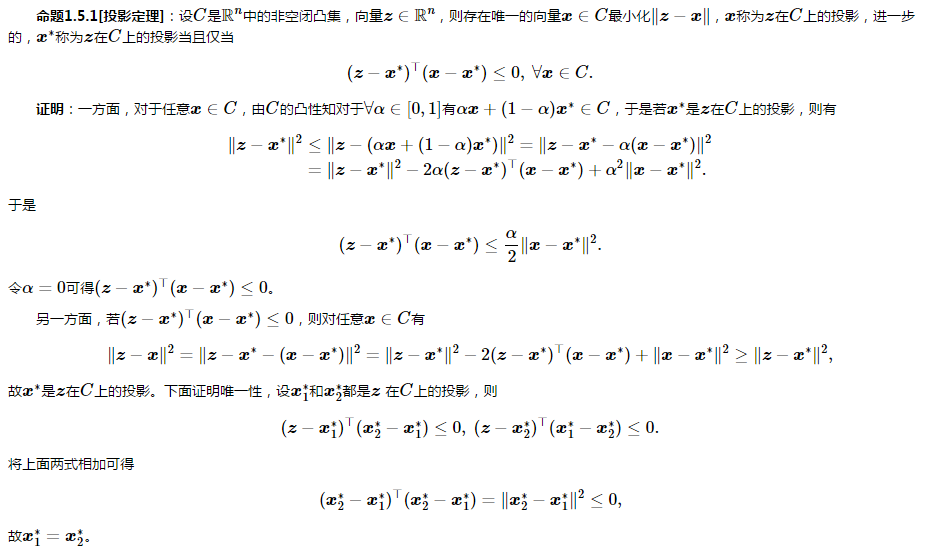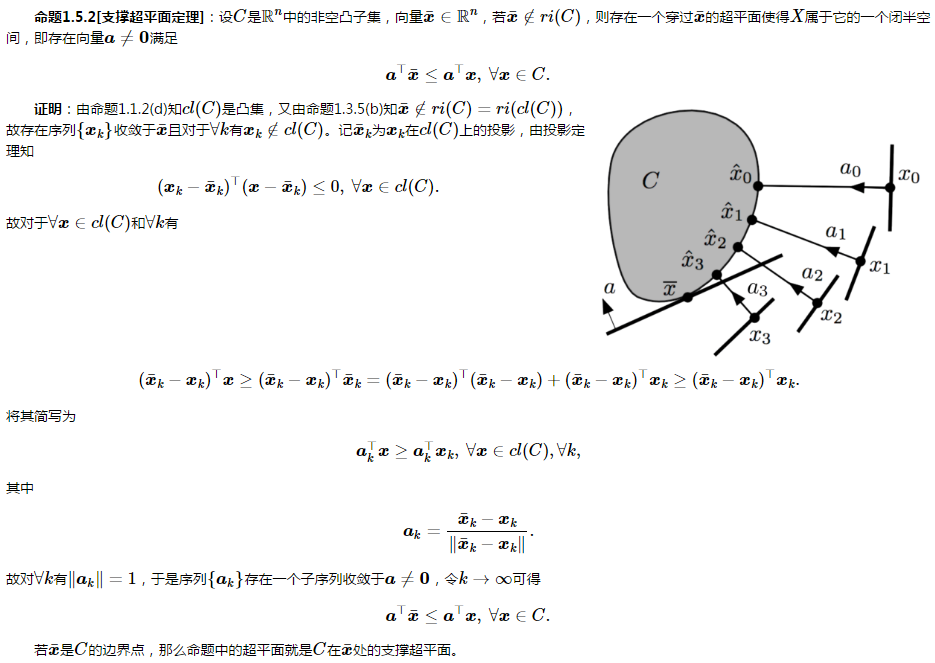展开全文SVM KKT条件 Slater条件 对偶问题
• 粒子群算法求解带约束优化问题 源码实现 python实现。你体验下粒子群算法是如何求解等式约束、不等式约束、上下限约束的问题。
算法原理
之前求解的无约束的问题。 粒子群算法求解无约束优化问题 源码实现
算法原理如下今天讲解下求解约束优化的问题。该问题常用的方法是罚函数法。即如果一个解x不满足约束条件，就对适应度值设置一个惩罚项。它的思想类似线性规划内点法，都是通过增加罚函数，迫使模型在迭代计算的过程中始终在可行域内寻优。 假设有如下带约束的优化问题问题中既含有等式约束，也含有不等式约束，当一个解x不满足约束条件时，则会对目标函数增加惩罚项，这样就把带约束优化问题变成无约束优化问题，新的目标函数如下：其中σ是惩罚因子，一般取σ=t√t ，P(x)是整体惩罚项，P(x)的计算方法如下：
对于不等式gi(x)<=0,惩罚项即如果gi(x)<=0,则ei(x)=0,否则ei(x)=-gi(x)  对于等式约束需要先转换成不等式约束，一个简单的方法设定一个等式约束容忍度值ε,新的不等式约束为因此等式约束的惩罚项为整体惩罚性P(x)是各个约束惩罚项的和，即：由于约束条件之间的差异，某些约束条件可能对个体违反程度起着决定性的作用，为了平衡这种差异，对惩罚项做归一化处理，下面的公式推导将不区分等式约束和不等式约束，在实际处理中做区分即可：其中Lj表示每个约束条件的违背程度，m为约束条件的个数。公式中分子的意思是，对每个粒子xi计算违反第j个约束的惩罚项，然后求和；分母的意思：对每个粒子xi计算违背每个约束的惩罚项，然后求和，因此Lj也可以看成是第j个约束惩罚项的权重。 最后得到的粒子xi的整体惩罚项P(x)的计算公式：在粒子群算法中，每一步迭代都会更新Pbest和Gbest，虽然可以将有约束问题转换为无约束问题进行迭代求解，但是问题的解xi依然存在不满足约束条件的情况，因此需要编制一些规则来比较两个粒子的优劣，规则如下：
如果两个粒子xi和xj都可行，则比较其适应度函数f(xi)和f(xj)，值小的粒子为优。当两个粒子xi和xj都不可行，则比较惩罚项P(xi)和P(xj），违背约束程度小的粒子更优。当粒子xi可行而粒子xj不可行，选可行解。
对于粒子的上下限约束 可以体现在位置更新函数里，不必加惩罚项。 具体思路就是遍历每一个粒子的位置，如果超除上下限，位置则更改为上下限中的任何一个位置
算例
语言python3.7 问题如下：此函数图像为方便粒子群算法的迭代写代码，肯定要如下格式矩阵，用来存储粒子群每个粒子xi的历史最优位置Pbest、总的适应度值fitness、目标函数的值、约束1的惩罚项e1、约束2的惩罚项e2
粒子序号Pbest_fitnessPbest_efitnessfe1e2ex1l历史最优位置对应的适应度历史最优位置对应的惩罚项当前的适应度fitness=f+e当前目标函数值约束1的惩罚项e1约束2的惩罚项e2惩罚项的和e=L1e1+L2e2x2…
步骤1：初始化参数
import numpy as np
import matplotlib.pyplot as plt
import matplotlib as mpl

mpl.rcParams['font.sans-serif'] = ['SimHei']  # 指定默认字体
mpl.rcParams['axes.unicode_minus'] = False  # 解决保存图像是负号'-'显示为方块的问题

# PSO的参数
w = 1  # 惯性因子，一般取1
c1 = 2  # 学习因子，一般取2
c2 = 2  #
r1 = None  # 为两个（0,1）之间的随机数
r2 = None
dim = 2  # 维度的维度#对应2个参数x,y
size = 100  # 种群大小，即种群中小鸟的个数
iter_num = 1000  # 算法最大迭代次数
max_vel = 0.5  # 限制粒子的最大速度为0.5
fitneess_value_list = []  # 记录每次迭代过程中的种群适应度值变化


步骤2：这里定义一些参数，分别是计算适应度函数和计算约束惩罚项函数

def calc_f(X):
"""计算粒子的的适应度值，也就是目标函数值，X 的维度是 size * 2 """
A = 10
pi = np.pi
x = X
y = X
return 2 * A + x ** 2 - A * np.cos(2 * pi * x) + y ** 2 - A * np.cos(2 * pi * y)

def calc_e1(X):
"""计算第一个约束的惩罚项"""
e = X + X - 6
return max(0, e)

def calc_e2(X):
"""计算第二个约束的惩罚项"""
e = 3 * X - 2 * X - 5
return max(0, e)

def calc_Lj(e1, e2):
"""根据每个粒子的约束惩罚项计算Lj权重值，e1, e2列向量，表示每个粒子的第1个第2个约束的惩罚项值"""
# 注意防止分母为零的情况
if (e1.sum() + e2.sum()) <= 0:
return 0, 0
else:
L1 = e1.sum() / (e1.sum() + e2.sum())
L2 = e2.sum() / (e1.sum() + e2.sum())
return L1, L2

步骤3：定义粒子群算法的速度更新函数，位置更新函数

def velocity_update(V, X, pbest, gbest):
"""
根据速度更新公式更新每个粒子的速度
种群size=20
:param V: 粒子当前的速度矩阵，20*2 的矩阵
:param X: 粒子当前的位置矩阵，20*2 的矩阵
:param pbest: 每个粒子历史最优位置，20*2 的矩阵
:param gbest: 种群历史最优位置，1*2 的矩阵
"""
r1 = np.random.random((size, 1))
r2 = np.random.random((size, 1))
V = w * V + c1 * r1 * (pbest - X) + c2 * r2 * (gbest - X)  # 直接对照公式写就好了
# 防止越界处理
V[V < -max_vel] = -max_vel
V[V > max_vel] = max_vel
return V

def position_update(X, V):
"""
根据公式更新粒子的位置
:param X: 粒子当前的位置矩阵，维度是 20*2
:param V: 粒子当前的速度举着，维度是 20*2
"""
X=X+V#更新位置
size=np.shape(X)#种群大小
for i in range(size):#遍历每一个例子
if X[i]<=1 or X[i]>=2:#x的上下限约束
X[i]=np.random.uniform(1,2,1)#则在1到2随机生成一个数
if X[i] <= -1 or X[i] >= 0:#y的上下限约束
X[i] = np.random.uniform(-1, 0, 1)  # 则在-1到0随机生成一个数
return X


步骤4：每个粒子历史最优位置更优函数，以及整个群体历史最优位置更新函数，和无约束约束优化代码类似，所不同的是添加了违反约束的处理过程

def update_pbest(pbest, pbest_fitness, pbest_e, xi, xi_fitness, xi_e):
"""
判断是否需要更新粒子的历史最优位置
:param pbest: 历史最优位置
:param pbest_fitness: 历史最优位置对应的适应度值
:param pbest_e: 历史最优位置对应的约束惩罚项
:param xi: 当前位置
:param xi_fitness: 当前位置的适应度函数值
:param xi_e: 当前位置的约束惩罚项
:return:
"""
# 下面的 0.0000001 是考虑到计算机的数值精度位置，值等同于0
# 规则1，如果 pbest 和 xi 都没有违反约束，则取适应度小的
if pbest_e <= 0.0000001 and xi_e <= 0.0000001:
if pbest_fitness <= xi_fitness:
return pbest, pbest_fitness, pbest_e
else:
return xi, xi_fitness, xi_e
# 规则2，如果当前位置违反约束而历史最优没有违反约束，则取历史最优
if pbest_e < 0.0000001 and xi_e >= 0.0000001:
return pbest, pbest_fitness, pbest_e
# 规则3，如果历史位置违反约束而当前位置没有违反约束，则取当前位置
if pbest_e >= 0.0000001 and xi_e < 0.0000001:
return xi, xi_fitness, xi_e
# 规则4，如果两个都违反约束，则取适应度值小的
if pbest_fitness <= xi_fitness:
return pbest, pbest_fitness, pbest_e
else:
return xi, xi_fitness, xi_e

def update_gbest(gbest, gbest_fitness, gbest_e, pbest, pbest_fitness, pbest_e):
"""
更新全局最优位置
:param gbest: 上一次迭代的全局最优位置
:param gbest_fitness: 上一次迭代的全局最优位置的适应度值
:param gbest_e:上一次迭代的全局最优位置的约束惩罚项
:param pbest:当前迭代种群的最优位置
:param pbest_fitness:当前迭代的种群的最优位置的适应度值
:param pbest_e:当前迭代的种群的最优位置的约束惩罚项
:return:
"""
# 先对种群，寻找约束惩罚项=0的最优个体，如果每个个体的约束惩罚项都大于0，就找适应度最小的个体
pbest2 = np.concatenate([pbest, pbest_fitness.reshape(-1, 1), pbest_e.reshape(-1, 1)], axis=1)  # 将几个矩阵拼接成矩阵 ，4维矩阵（x,y,fitness,e）
pbest2_1 = pbest2[pbest2[:, -1] <= 0.0000001]  # 找出没有违反约束的个体
if len(pbest2_1) > 0:
pbest2_1 = pbest2_1[pbest2_1[:, 2].argsort()]  # 根据适应度值排序
else:
pbest2_1 = pbest2[pbest2[:, 2].argsort()]  # 如果所有个体都违反约束，直接找出适应度值最小的
# 当前迭代的最优个体
pbesti, pbesti_fitness, pbesti_e = pbest2_1[0, :2], pbest2_1[0, 2], pbest2_1[0, 3]
# 当前最优和全局最优比较
# 如果两者都没有约束
if gbest_e <= 0.0000001 and pbesti_e <= 0.0000001:
if gbest_fitness < pbesti_fitness:
return gbest, gbest_fitness, gbest_e
else:
return pbesti, pbesti_fitness, pbesti_e
# 有一个违反约束而另一个没有违反约束
if gbest_e <= 0.0000001 and pbesti_e > 0.0000001:
return gbest, gbest_fitness, gbest_e
if gbest_e > 0.0000001 and pbesti_e <= 0.0000001:
return pbesti, pbesti_fitness, pbesti_e
# 如果都违反约束，直接取适应度小的
if gbest_fitness < pbesti_fitness:
return gbest, gbest_fitness, gbest_e
else:
return pbesti, pbesti_fitness, pbesti_e


步骤5：PSO
流程图如图：约束体现在更新粒子最优 ，和全局最优上。
# 初始化一个矩阵 info, 记录：
# 0、种群每个粒子的历史最优位置对应的适应度，
# 1、历史最优位置对应的惩罚项，
# 2、当前适应度，
# 3、当前目标函数值，
# 4、约束1惩罚项，
# 5、约束2惩罚项，
# 6、惩罚项的和
# 所以列的维度是7
info = np.zeros((size, 7))

# 初始化种群的各个粒子的位置
# 用一个 20*2 的矩阵表示种群，每行表示一个粒子
X = np.random.uniform(-5, 5, size=(size, dim))

# 初始化种群的各个粒子的速度
V = np.random.uniform(-0.5, 0.5, size=(size, dim))

# 初始化粒子历史最优位置为当当前位置
pbest = X
# 计算每个粒子的适应度
for i in range(size):
info[i, 3] = calc_f(X[i])  # 目标函数值
info[i, 4] = calc_e1(X[i])  # 第一个约束的惩罚项
info[i, 5] = calc_e2(X[i])  # 第二个约束的惩罚项

# 计算惩罚项的权重，及适应度值
L1, L2 = calc_Lj(info[i, 4], info[i, 5])
info[:, 2] = info[:, 3] + L1 * info[:, 4] + L2 * info[:, 5]  # 适应度值
info[:, 6] = L1 * info[:, 4] + L2 * info[:, 5]  # 惩罚项的加权求和

# 历史最优
info[:, 0] = info[:, 2]  # 粒子的历史最优位置对应的适应度值
info[:, 1] = info[:, 6]  # 粒子的历史最优位置对应的惩罚项值

# 全局最优
gbest_i = info[:, 0].argmin()  # 全局最优对应的粒子编号
gbest = X[gbest_i]  # 全局最优粒子的位置
gbest_fitness = info[gbest_i, 0]  # 全局最优位置对应的适应度值
gbest_e = info[gbest_i, 1]  # 全局最优位置对应的惩罚项

# 记录迭代过程的最优适应度值
fitneess_value_list.append(gbest_fitness)
# 接下来开始迭代
for j in range(iter_num):
# 更新速度
V = velocity_update(V, X, pbest=pbest, gbest=gbest)
# 更新位置
X = position_update(X, V)
# 计算每个粒子的目标函数和约束惩罚项
for i in range(size):
info[i, 3] = calc_f(X[i])  # 目标函数值
info[i, 4] = calc_e1(X[i])  # 第一个约束的惩罚项
info[i, 5] = calc_e2(X[i])  # 第二个约束的惩罚项
# 计算惩罚项的权重，及适应度值
L1, L2 = calc_Lj(info[i, 4], info[i, 5])
info[:, 2] = info[:, 3] + L1 * info[:, 4] + L2 * info[:, 5]  # 适应度值
info[:, 6] = L1 * info[:, 4] + L2 * info[:, 5]  # 惩罚项的加权求和
# 更新历史最优位置
for i in range(size):
pbesti = pbest[i]
pbest_fitness = info[i, 0]
pbest_e = info[i, 1]
xi = X[i]
xi_fitness = info[i, 2]
xi_e = info[i, 6]
# 计算更新个体历史最优
pbesti, pbest_fitness, pbest_e = \
update_pbest(pbesti, pbest_fitness, pbest_e, xi, xi_fitness, xi_e)
pbest[i] = pbesti
info[i, 0] = pbest_fitness
info[i, 1] = pbest_e
# 更新全局最优
pbest_fitness = info[:, 2]
pbest_e = info[:, 6]
gbest, gbest_fitness, gbest_e = \
update_gbest(gbest, gbest_fitness, gbest_e, pbest, pbest_fitness, pbest_e)
# 记录当前迭代全局之硬度
fitneess_value_list.append(gbest_fitness)

# 最后绘制适应度值曲线
print('迭代最优结果是：%.5f' % calc_f(gbest))
print('迭代最优变量是：x=%.5f, y=%.5f' % (gbest, gbest))
print('迭代约束惩罚项是：', gbest_e)

#迭代最优结果是：1.00491
#迭代最优变量是：x=1.00167, y=-0.00226
#迭代约束惩罚项是： 0.0
# 从结果看，有多个不同的解的目标函数值是相同的，多测试几次就发现了

# 绘图
plt.plot(fitneess_value_list[: 30], color='r')
plt.title('迭代过程')
plt.show()作者：电气 余登武展开全文算法 python
• 约束优化进化算法主要研究如何利用进化计算方法求解约束优化问题,是进化计算领城的个重要研究课题.约束优化问题求解存在约束区域离散、等式约束、非线性约束等挑战,其问题的本质是,如何处理可行解与不可行解的关系...


约束优化进化算法综述

1.摘要
约束优化进化算法主要研究如何利用进化计算方法求解约束优化问题,是进化计算领城的一个重要研究课题.约束优化问题求解存在约束区域离散、等式约束、非线性约束等挑战,其问题的本质是,如何处理可行解与不可行解的关系才能使得算法更高效.首先介绍了约束优化问题的定义;然后,系统地分析了目前存在的约束优化方法;同时,基于约束处理机制,将这些方法分为罚函数法、可行性法则、随机排序法、ᴈ-约束处理法、多目标优化法、混合法等 6 类,并从约束处理方法的角度对约束优化进化算法的最新研究进展进行综述;最后,指出约束优化进化算法需进一步研究的方向与关键问题.
2.介绍
罚函数法通过添加惩罚因子的方式将约束优化问题转化为无约束优化问题,是最为传统的约束处理方法之一,该类方法的不足在于惩罚参数的选取比较困难可行性法则是 Deb 提出的一种约束处理方法,其将可行解和不可行解分离处理,并且可行解的适应度函数值优于不可行解的适应度函数值,因此,该方法使得不可行解难以进入种群,这样就使得该方法在求解最优解位于可行域边界上的约束优化问题时可能存在问题随机排序法采用冒泡排序的方法来处理约束优化问题;ᴈ-约束处理法通过设定ᴈ值将约束划分为不同的区间,个体之间的比较在不同的约束区间内采用不同的处理方法,该方法是对可行性法则的扩展,它以牺牲时间为前提,对搜索空间进行充分搜索多目标优化法根据将约束优化问题转换为多目标优化问题,并采用多目标优化技术来处理问题,然而,没有引入偏好的多目标优化方法可能并不像人们所想象的那样高效混合法求解约束优化问题是近年来研究的热点,该方法通过将不同的约束处理机制相结合的方法来处理约束优化问题.
3.约束处理方法详细介绍
3.1罚函数法
静态罚函数
Constrained optimization via genetic algorithms. Simulation Trans. of the Society for Modeling & Simulation International----1994，该方法将约束违反的程度分为多个等级,不同等级采用不同的惩罚系数 ,约束违反程度越大,则违反等级越高,对应的惩罚力度也就越大.分级处理机制相对单一数值法更为合理,但该方法需要一定的先验信息来确定各等级的惩罚系数值,另外还产生了较多需要调节的参数.这类方法实现相对简单且多用于求解简单的约束优化问题,不足之处在于它的通用性,因为它对每一类问题,都需要进行惩罚系数的反复实验,寻找到合适的惩罚系数.
动态罚函数
On the use of non-stationary penalty functions to solve nonlinear constrained optimization problems with GA’s----1994，该方法不需要设定惩罚系数值,但其他参数仍需要设定.与静态罚函数法相比,虽然动态罚函数法在性能方面上更具优越性,但是找到理想的惩罚系数动态变化规律(初始化惩罚系数的选取)仍然是一个棘手的问题
死亡罚函数
Problem-Independent handling of constraints by use of metric penalty functions----1996，拒绝不可行解
退火罚函数法
Evolutionary optimization of constrained problems----1994，惩罚系数随着温度的降低而不断增加
隔离遗传算法
A segregated genetic algorithm for constrained structural optimization----1995，通过两个不同的惩罚系数来对群体中的个体进行评价,其旨在于在过大和过小的惩罚系数之间达到平衡
协同罚函数法
Use of a self-adaptive penalty approach for engineering optimization problems----2000，包括两个群体,第 1 个群体 中个体表示问题的解,第 2 个群体的个体为惩罚系数的集合,利用它来计算第 1 个群体中个体的适应度值An effective co-evolutionary differential evolution for constrained optimization----2006，包括两个群体,第 1 个群体中个体表示问题的解,第 2 个群体的个体为惩罚系数的集合,利用它来计算第 1 个群体中个体的适应度值
自适应罚函数
New results using adaptive segregational constraint handling----2002，采用进化策略作为搜索框架,为保持种群中可行解与不可行解的平衡,始终允许在种群中保留不可行个体以增强全局搜索能力,算法根据种群中可行解在整个种群中所占的比例来动态调整惩罚系数Self-Adaptive fitness formulation for constrained optimization----2003，一种自适应适应值表示法,该方法将惩罚分为两个阶段进行,都是通过提取种群中的边界个体的信息来确定惩罚强度A genetic algorithm for solving multi-constrained function optimization problems based on KS function----2007，一种遗传算法求解多约束优化问题,它利用 KS 函数的融合特征将多个约束条件融合成一个约束条件,从而减少了静态罚函数法中惩罚系数数量过多的问题和避免了约束条件权重分配问题A rough penalty genetic algorithm for constrained optimization----2013，一个高效的遗传算法Adaptive penalty and barrier function based on fuzzy logic----2015，一个高效的遗传算法A rough penalty genetic algorithm for constrained optimization. Information Sciences----2013，一种自适应惩罚函数的方法,该方法采用当前种群中可行解的比例来自适应地控制惩罚的强度.与其他方法不同的是:该方法除了引入距离项(把种群中的个体映射到二维坐标系内的点,而该点到坐标原点的距离)之外,还为了找到最佳不可行解,对不可行解采用双惩罚.该方法旨在于先挖掘出种群中具有较好质量的不可行解,然后再引导种群向可行域或者最优解逼近Variants of an adaptive penalty scheme for steady-state genetic algorithms in engineering optimization. Engineering Computations----2015，一种自适应惩罚函数方法,并将其和稳态遗传算法相结合,用于解决工程优化问题.该方法为每个约束条件分配各自的惩罚系数,这样来实现约束条件之间的平衡.在进化的过程中,该方法根据种群状态信息(比如种群中是否存在可行解和每个约束的违反程度)自适应地决定每个约束条件的惩罚系数的变化A modified covariance matrix adaptation evolution strategy with adaptive penalty function and restart for constrained optimization----2014，一种自适应罚函数法,并将其与改进的自适应协方差矩阵进化策略(CMA-ES)结合起来,得到了一个新的约束优化算法.在自适应罚函数法中,惩罚系数随着每一代群体中全部不可行个体的约束违反信息的变化而变化A CMA-ES-Based 2-Stage memetic framework for solving constrained optimization problems----2014，一种两阶段的文化基因框架:第 1 阶段将约束优化问题变成无约束优化问题进行处理,其目标是为局部搜索阶段找到好的初始个体,为了实现这个目标,采用自适应惩罚 CMA-ES 作为全局搜索策略;第 2 阶段使用局部搜索策略(比如单形法)来进一步改善解的质量,其目标是为了找到全局最优解.该方法用于解决规模大的约束优化问题具有很好的优化效果.Mukherjee S. A fuzzy rule-based penalty function approach for constrained evolutionary optimization----2014，一种模糊惩罚法(fuzzy rule-based penalty function approach,简称 FRSP),并将该模糊惩罚法与差分进化算法结合,用来解决约束优化问题,它具有两个惩罚项,其中:第 1 项惩罚采用了基于条件法则的 Mamdani 模糊推理系统,该模型事先掌握了自适应函数所有必需的条件(作为条件库),然后通过模糊逻辑控制(FLC),将个体的约束违反程度、目标函数和种群中可行解的比例作为模糊输入,经过模糊推理过程后,最终得到模糊输出量,即惩罚值;第 2 项惩罚项是根据种群中可行解所占百分比来动态地平衡目标函数和约束条件Constraint Handling in Multiobjective Evolutionary Optimization----2008，跟踪当前人口中可行解决方案的百分比，以确定要添加的惩罚金额。 一小部分可行的个人导致更大的惩罚，而更大的百分比产生一个小的惩罚因素。 该技术能够从可行和不可行的解中平衡信息。
3.2可行性法则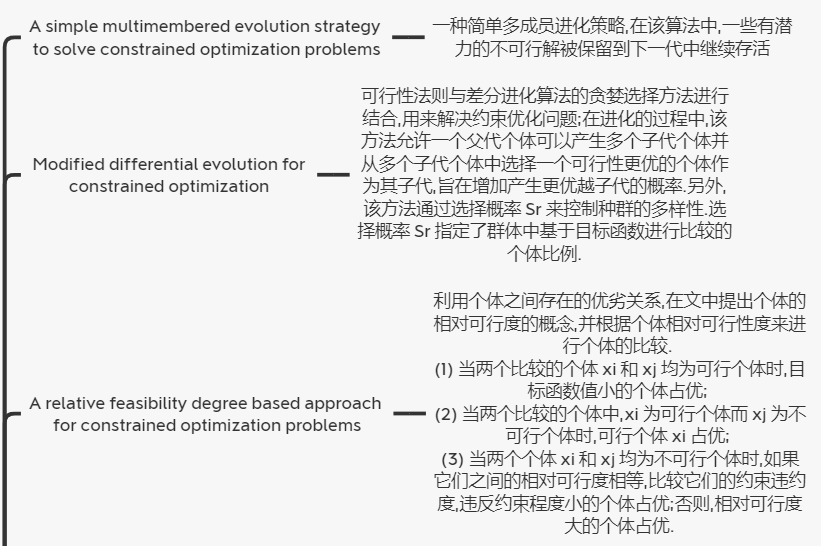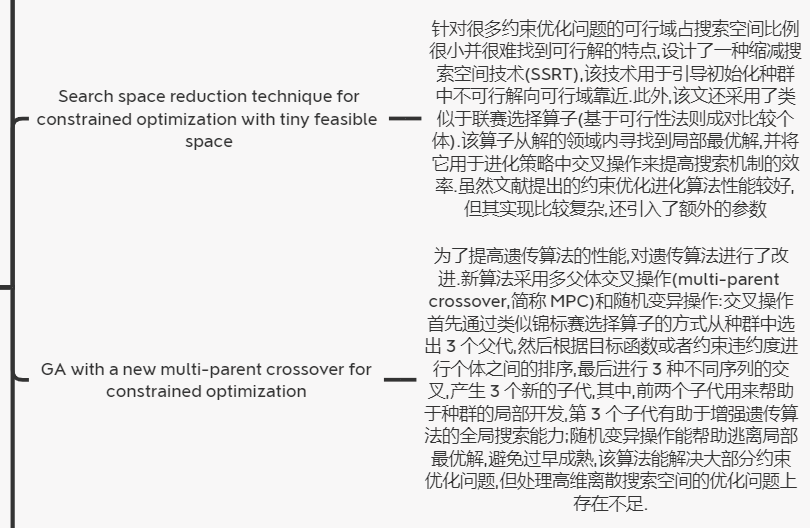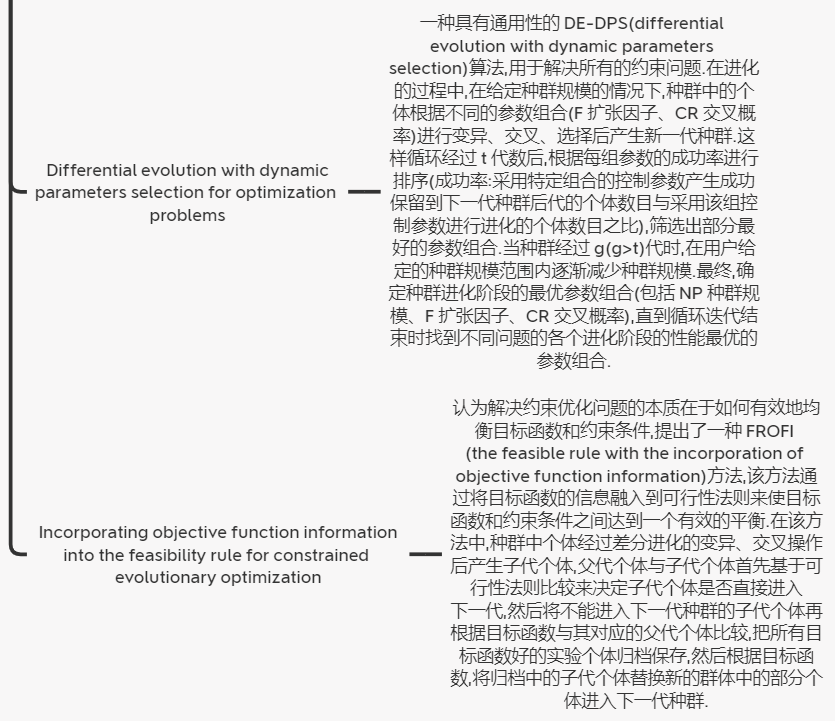3.3随机排序法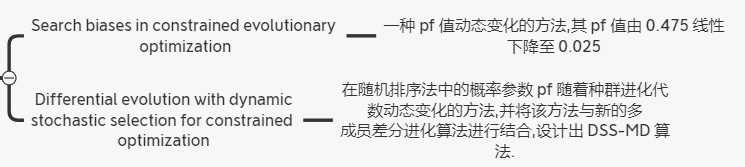3.4ᴈ约束处理法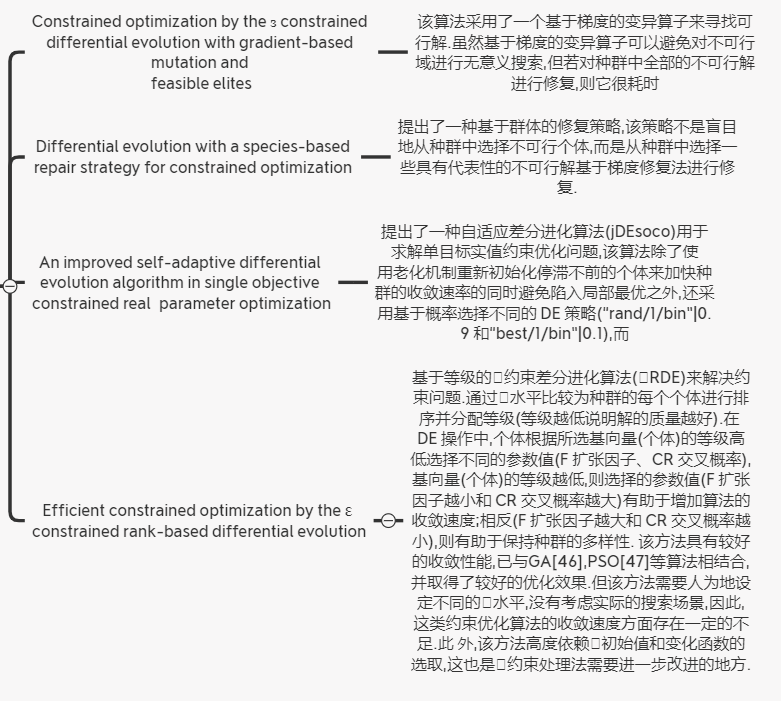3.5多目标优化法
Constrained optimization by multi-objective genetic algorithm----1997，提出了 COMOGA(constrained optimization by multi-objective genetic algorithm)算法,在该算法中,约束优化问题被视作约束满足问题或无约束优化问题来处理.当约束优化问题被视为约束满足问题时,目标函数被忽略,此时,个体之间的比较由 Pareto 排序决定,Pareto 排序基于约束违反来定义.当约束优化问题被视为无约束优化问题时,约束条件被忽略,此时,个体之间的比较由目标函数决定.Treating constraints as objectives for single-objective evolutionary optimization----2000，基于向量评估遗传算法求解约束优化问题.将种群分成相等规模的子种群,每个子群对应一个约束条件,其中,某个子群体将目标函数作为适应度函数来评价个体,其余的子群体将相应的单个约束条件作为适应度函数来评价个体,其目的在于:每个子群体试图找到对应约束条件的可行解,通过联合所有的子种群,该方法找到所有约束条件下的可行解.该算法的主要缺陷在于:子群体的个数会随着约束条件的个数呈线性增加;另外,如何确定子群体的合理规模有待进一步研究.A generic framework for constrained optimization using genetic algorithms----2005，提出了一种包含两个阶段的通用框架来处理约束问题:第 1 阶段,当种群里没有可行解时,该方法仅使用约束违反程度选择个体;当种群中出现可行解时(第 2 阶段),原来的约束优化问题转化为一个双目标优化问题,其中,一个目标是原来的目标函数,而另外一个目标则是约束违反程度,之后,再使用 Pareto 排序的方法来选择个体.Multi-Objective optimization and hybrid evolutionary algorithm to solve constrained optimization problems. IEEE Trans. on Systems, Man, and Cybernetics----2007，提出了一种 HCOEA(hybrid onstrained optimization evolutionary algorithm)算法,在该算法中,将约束问题转化为双目标优化问题,采用带小生境操作的遗传算法框架,并基于非支配解的概念进行解之间的替换,但只有子代个体在支配父代个体时,才以概率 1替换父代个体.同时,算法除了引入类似于 MOEA中不可行解的存储和替换机制外,还引入一种聚类分割和多个父代交叉的局部搜索技术,根据搜索空间中各个个体之间的相互距离,将种群聚类为 M 个子种群,各子种群通过内部交叉产生新个体,进而组合新种群.A multiobjective differential evolution algorithm for constrained optimization----2008，提出了一种多目标差分进化算法,该算法引入正交设计法用于种群的初始化以及基于正交的交叉算子来提高算法的局部搜索能力,并采用差分进化作为搜索机制.在该算法中,比较父代个体和子代个体时先优考虑约束条件.如果子代个体 Pareto 支配父代个体,则立即用子代个体取代父代个体.如果两个比较个体在约束条件上存在非支配关系,则再考虑目标函数和约束违约度,被支配的父代个体则立即被子代个体取代.之 后,将非劣的子代个体与父代个体组成一个混合群体,然后选取其中的非劣个体进入下一代.Constrained optimization evolutionary algorithms----2009，对该类算法进行了系统的分析与论述Multiobjective optimization algorithm for solving constrained single objective problems----2010,提出了一种 sp-MODE(the multiobjective differential evolution algorithm with spherical pruning)法来解决高约束优化问题,该方法基于双重选择策略.在每一次迭代,首先从种群§中随机选择 N 个个体组成的子群体通过 DE 进化产生子代群体(Pn)后,然后利用 Pareto 排序的方法从联合群体(Pn ∪D)中找出所有的非劣个体,最后采用球形修剪技术从非劣解集中筛选出每个球形网格内具有较小约束违反程度的非劣个体(D).同时,球形裁剪技术维持了种群的多样性.当产生一个新的个体时,Pareto 占优用于比较该个体与其父代个体的优劣Performance of infeasibility empowered memetic algorithm for CEC 2010 constrained optimization problems----2010,针对很多约束优化问题的最优解位于可行域边界的特点,提出了 IDEA(infeasibility driven evolutionary algorithm)法.在该方法中,约束优化问题被转化为两个目标的多目标优化问题来处理:第 1个目标为原目标函数 f(x);另外一个目标为基于种群成员之间的个体相对约束违约度(根据每个约束条件的违反程度,将种群中的每个个体从低到高进行排序;同时,约束违反度越低的个体分配的等级越高(可行个体对应的等级为 0),个体相对约束违约度是所有约束条件上对应等级的总和).在进化的过程,IDEA 首先根据个体的可行性将种群划分成两个群体(可行解集和不可行解集),并分别对该两个群体使用 Pareto 排序过程的方法来定义个体的等级,然后再按一定比例 u(用户定义),分别从两个群体中选择个体组成下一代种群.Combining multiobjective optimization with differential evolution to solve constrained optimization problems----2012,提出 CMODE(combining multiobjective optimization with differential evolution)算法,该算法将多目标优化策略与差分进化算法结合来处理约束问题.CMODE 首先从种群中随机选择 n 个个体组成的群体经过差分进化的变异、交叉操作产生子代群体,然后从子代群体找出全部的非劣个体;接着,随机替换父代群体中的任意一个被支配个体(如果该劣于个体存在).此外,该方法采用一种不可行解存档和替换机制,将每一代种群中好的不可行解存档起来,用于在后续进化中随机地替换种群中的个体.一方面,该机制保证了种群的多样性,使算法不会过早收敛到某些可行的局部最优;另一方面,该机制有利于对边界区域进行局部搜索.A dynamic hybrid framework for constrained evolutionary optimization----2012,用来求解约束问题.DyHF 根据当前种群中可行解的比例自发地实行全局搜索模型或者局部搜索模型.局部搜索模型首先将种群通过聚类方法划分成多个群体,然后分别从每个子代群体中找出所有的非劣个体,最后选择一个非劣个体随机地替换其父代群体中一个劣于个体(如果该个体存在),使种群从不同方向迫近可行域.全局搜索模型是从整个种群的角度利用 Pareto 优劣关系比较个体,选择父代个体和子代个体中的优胜者.Biased multiobjective optimization for constrained single-objective evolutionary optimization----2014,提出了一种有偏支配的约束处理技术,使用该有偏支配进行个体的比较.假设第 k 个目标函数 fk(x)是偏向的目标函数(有偏目标函数),有偏支配的定义A dual-population differential evolution with coevolution for constrained optimization----2014,提出了一种双种群协同进化差分算法,它把原来的约束问题转变成一个双目标优化问题,其 中,第 1 个目标是目标函数,第 2 个目标是约束违约度.在进化过程中,为了分别对待这两个目标,整个种群根据个体的可行性被划分成两个子群体,每个子群体优化对应的目标.同时,该文还引用了一种信息共享机制促进两个子群体之间搜索信息的相互交流,旨在让不可行个体变成可行个体并寻找到最优解.
3.6混合法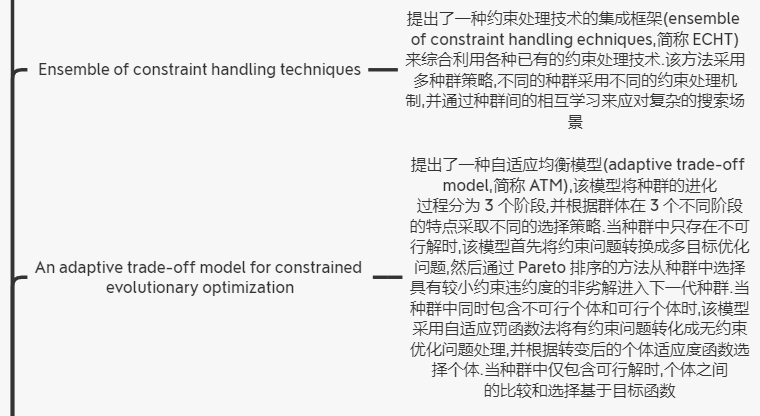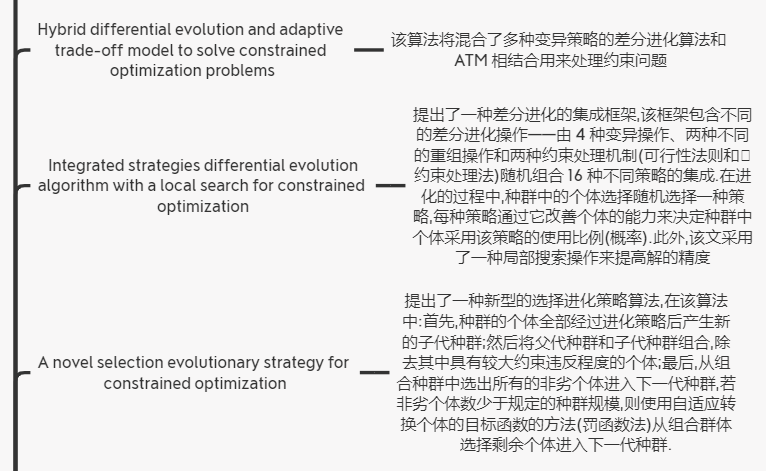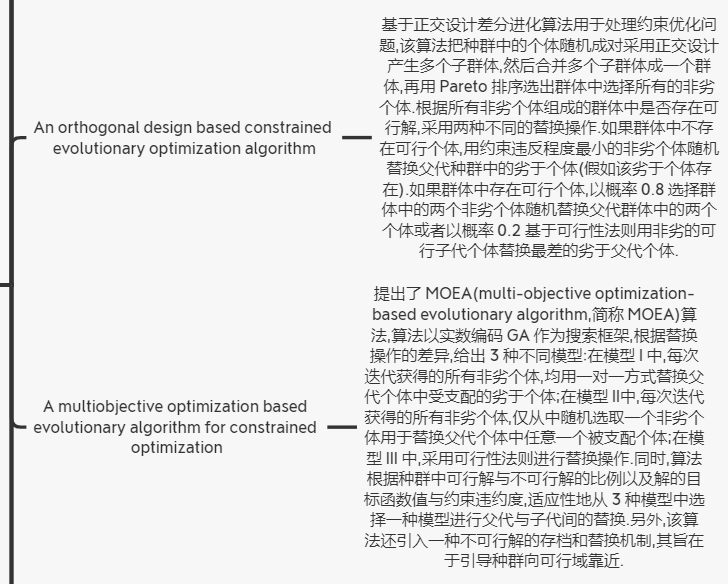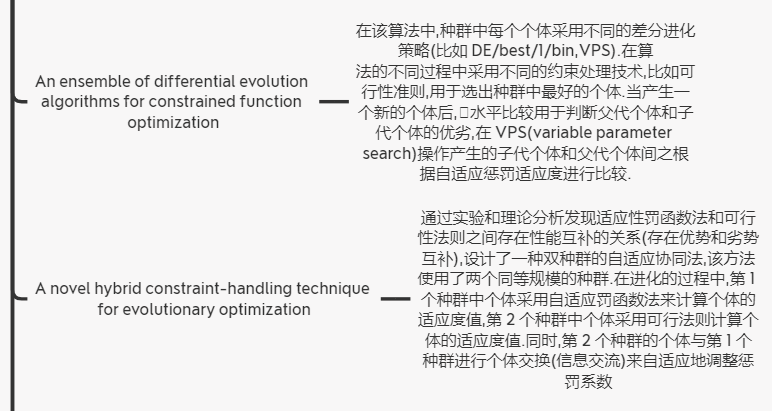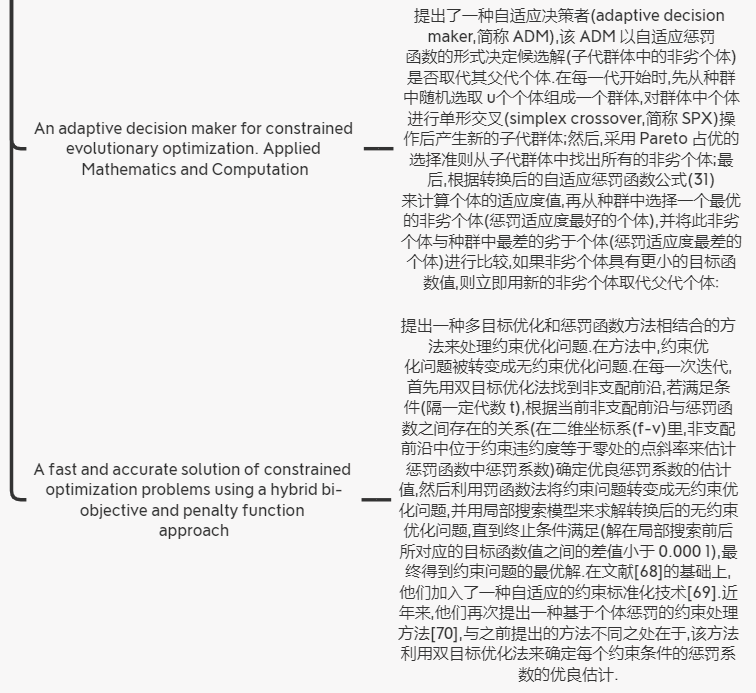4.亟待解决的问题
虽然约束优化进化算法已经取得了一定的成果,但是仍有很多问题需要解决,主要是: (1) 等式约束处理 目前,约束优化进化算法对于等式约束优化问题的约束效果并不令人满意,难以收敛算法最优解.目前主要采用动态缩小阈值值来提高算法的收敛性能,但面对非线性等式约束、离散等式约束等问题,依然具有较大的挑战. (2) 离散约束优化问题 在该类约束优化问题中,问题的可行区域是离散的,这使得算法容易陷入某个可行区域中的最优解,难以获得全局最优解.如何使得搜索能穿梭于各个可行区域间,是该问题的主要挑战.多种群、分布估计可能是解决该 问题的可行方案之一. (3) 约束条件与优化目标之间的不平衡 约束优化不仅要求所得解的质量,同时要求满足约束条件.对于有些情况,如过分强调满足约束条件,将有可能导致搜索偏离性能好的区域;相反,如果过分强调解的质量,则有可能导致搜索空间偏离可行域.因此,如何根据问题的先验信息和从搜索过程中得到的知识来有效协调约束与目标之间的不平衡非常重要. (4) 可行解与不可行解的分别对待 约束优化问题相对于普通约束优化问题,其本质区别在于如何对待不可行解携带的信息.在早期的研究中,对该问题的研究取得了较大的进展,但近年来对该基本问题并没有取得实质性的进展.这主要是由于近年来进化算法的搜索效率得到了快速的发展,弥补了约束处理的不足.模糊理论、粗糙理论、ᴈ约束处理等技术可能是该问题的研究方向. (5) 普适的约束处理技术 目前,不同的约束处理技术对应不同的随机搜索技术有着不同的搜索效率.无免费的午餐定理告诉我们,没有任何算法能对所有问题都高效.但我们能不能设计一种对大多数类别算法都高效的约束处理机制?这将会是未来研究的方向之一. (6) 自适应约束处理技术 正如前面所说,不同的约束处理技术对应不同的随机搜索技术有着不同的搜索效率.设计一种针对不同的搜索状态而采用不同的约束处理机制,自适应地选择不同的约束处理技术可能是可行的方案之一.另外,超启发式框架也具有一定的可行性.
5.总结
本文对受约束的多目标问题研究现状进行了详细总结，并重点分析了6大类约束处理方法

返回受约束的多目标优化问题优秀论文及总结目录

展开全文• 这篇论文，作者提出了个序列感知推荐模型。该模型利用自注意力机制从用户历史交互中推测出item-item之间的关系。通过自注意力机制可以在用户交互轨迹中评估每个item的相关权重，以便更好的来学习用户瞬...


Next Item Recommendation with self-Attention
摘要关键词介绍相关工作1.序列感知推荐系统（sequence-aware）2.基于深度神经网络的推荐3.神经注意力模型
模型：AttRec1.序列推荐2.使用自注意力机制建模短期意图3用户长期喜好建模4.模型训练

ACM 2018
原文地址

摘要
这篇论文，作者提出了一个序列感知推荐模型。该模型利用自注意力机制从用户历史交互中推测出item-item之间的关系。通过自注意力机制可以在用户交互轨迹中评估每个item的相关权重，以便更好的来学习用户瞬时兴趣的表示。这个模型最后训练在**度量学习框架（metric learning framework）**上，并且考虑了用户短期和长期的意图。在不同领域大量的数据集上的实验证明了，我们的方法表现了最有的性能。
关键词
推荐系统、序列推荐、自注意力
介绍
在很多领域有丰富的历史交互数据存在，比如点击率、购买记录、浏览等，通过这些数据可以构建有效的推荐系统。利用历史数据来推算出下一项推荐是很多基于机器学习的推荐系统基础。很多现有成果利用用户行为的再现和丰富的序列模型来预测用户的next item。这篇论文利用自回归趋势来建立高效的序列推荐系统。 近几年CNN和RNN对于解决序列问题方法很火热。RNN中，相连item之间的交互可以被循环矩阵捕获，长期依赖关系可以保存在循环记忆中。CNN通过对输入序列进行滑动参数化地转换，隐式得捕获交互。但是这样的推荐系统有一个缺点：它们不能通过历史数据显示得捕获item-item之间的交互。建模用户上下文历史中的item-item之间的关系，是非常必要的。因为理解单个item pairs之间的粒度关系（fine-grained）而不是简单地掩饰他们是十分重要的。我们假设为我们的模型提出归纳偏置，将会提高表示的质量，进而提高序列推荐系统的性能。 为此，我们提出了一种神经序列推荐系统，其中序列表示不仅通过建模consecutive item，而且通过建模当前窗口all user interactions来学习。因此我们的模型，可以说是一种local-global的方法。我们认为，基于自注意力机制的神经模型，通过全体用户的历史交互序列，显示得表达item-item之间的相互作用。这不仅能够使我们了解全局信息，而且还能了解连续的K个item项之间的短期信息。基于自匹配矩阵（self-matching matrix），我们学习关注相互作用的序列，以选择出最相关的item，来产生最终的用户表示。实验证明，我们的模型贼好。 我们的模型运用度量学习框架的形式，在训练过程中，用户的选择item和预期item之间的距离被拉进。这是第一个在序列推荐系统中运用自注意力机制度量学习的例子。我们的贡献有以下三个： 1.新颖的序列推荐系统：模型结合自注意力网络和度量嵌入模型来建模用户临时的和长期的意图。 2.我们的框架在12个完善的数据集上试验了，性能优秀。演示了序列建模中， 显示得表达了item-item之间的关系。 3.我们实现了广泛的超参数和消融研究。
相关工作
1.序列感知推荐系统（sequence-aware）
user-item的交互记录在时间戳中，积累的数据能够对时间动态建模，并为用户偏好提供证据。 前人对此研究： Koren et al. 将用户和item偏好视为随时间变化的函数，对item的瞬时流行度和用户时间倾向建模 Xiong et al. 引入额外的时间因子，建立贝叶斯概率张量因子分解方法来模拟时间漂移 Wu et al.  使用递归神经网络模拟评级的瞬时演变 都是专门用于预测评级的。 为了生成个性化推荐排名列表： Rendle et al.  将矩阵分解和马尔可夫链相结合 He et al.  基于相似性的方法于马尔可夫链相融合的顺序推荐方法，除此之外，度量嵌入也很好 Feng et al.  通过引入嵌入翻译的思想来改进该模型
2.基于深度神经网络的推荐
深度学习用在推荐系统很多领域，比如多层感知器在建模用户关系时可以引入非线性表示。卷积神经网络可用于物品和用户的特征提取，自编码器从side信息中学习显著信息来提高推荐质量，RNN建模时间。 在所有的推荐系统中，基于会话的推荐系统和我们系统的任务相似，但是也有些不一样。在基于会话的推荐系统中，用户身份未知，因此模型结构和学习过程是分散的（divergent）。深度学习的灵活性使得将不同的神经网络相结合可以形成强大的混合推荐系统。这归功于其非线性、强大的学习表示、和序列建模。 Wang 提出分层表示模型，去捕获用户总体偏好和时序行为。 Tang 提出卷积序列模型，去学习用户瞬时轨迹。其性能比CNN好。 总体来说，CNN和RNN需要从大量数据在学习才有意义，稀疏的数据集使模型学习变得很困难。
3.神经注意力模型
神经注意力模型和人类视觉很类似，它只学习关注最重要的部分。标准的注意力可以整合到CNN和RNN中来克服其不足。特别得，注意力机制使得RNN容易记住非常长期的依赖关系，可以帮助CNN将注意力集中在最重要的部分。 我们的论文是基于一种新的模型，自注意力机制。自注意力机制关注的是两个序列的协作学习和自我匹配（co-learning and self-matching）*。其中一个序列的注意力权重取决于另一个序列，反之亦然。它可以在序列学习中代替RNN和CNN，以更低的计算复杂度达到更高的精度。我们用自注意力机制建模序列依赖关系和用户短期行为。但值得注意的是，在基于上下文的推荐系统中，使用自注意力机制并不是那么简单，因此我们的论文好呀！
模型：AttRec
AttRec即self-attentive sequential recommendation，本模型由两部分组成。一部分是用于建模用户短期意图的自注意力机制，一部分是建模用户长期偏好的协作度量学习。
1.序列推荐
用户最近的交互反映了用户近期的需求和意图。因此为了更好的地理解用户的时间偏好，建模用户短期交互是一项重要的任务。本文使用自注意力机制来捕获序列并使用它来建模用户最近的交互轨迹。U表用户，I表item。其中|U|=M，|I|=N。我们使用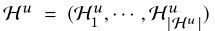表示用户u交互的item序列，是有序的。其中Hu属于I。序列推荐的目的是为了通过之前的轨迹，预测用户可能与之交互的下一项item。
2.使用自注意力机制建模短期意图
自注意力模型是注意力机制的一个特例，已成功应用于各自任务，它通过将单个序列与自身匹配来细化表示。在背景知识有限的情况下，自注意力可以保存上下文序列信息，并捕获序列中元素的关系。因此用自注意力来关注用户过去的行为。 自注意力机制的基础是被缩放的点积注意（dot-product attention）。该模型的输入由query、value、key三个组成，输出是value的加权和。其中权重矩阵由query和与其对应的key决定。在本文中，query、key、value三值相等，并且都是又用户最近的历史记录组成。假设用户的短期意图可以从她最近的交互L中得到。其中每一项都可以由d维的嵌入向量表示。X ∈ RN×d表明全体item的嵌入表示。最新的L项（比如从t-L+1到t）按顺序放在在如下矩阵中：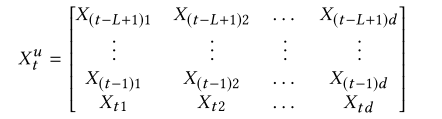最新的L项是Hu的子集。用户u的query、key、value在时间步长t下，等于Xut(X的上标是u下标是t）。 首先：我们将query和key通过共享参数的非线性变化整合到一个空间中。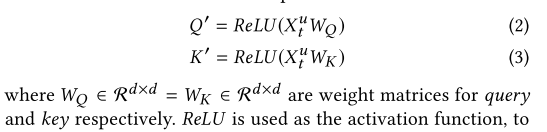ReLU是激活函数，是为了引入非线性表示到学习到的注意中。关联矩阵的计算如下：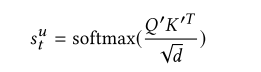输出是L*L的关联矩阵（或注意力map），表明L项之间的相似度。注意根号d被用于收缩点积注意。在我们这个例子中，d通过设得比较大（比如100）。这样的话，缩放因子可以减小非常小的梯度效应。在softmax之前应用屏蔽操作（屏蔽关联矩阵的对角线），以避免相同的query向量和key之间的高匹配分数。（不是很理解） 第二：我们保持Xut不变，在模型中使用恒等映射（identity mapping）（其他模型使用线性变换来映射）在其他应用领域中，value通常是预先训练的特征嵌入，例如字嵌入或图像特征。但本模型value由需要学习的参数构成，线性或非线性变换的加入将增加实际参数显露的难度。key和query是辅助信息，以便其不像value那样那么容易被转换。 最后，关联矩阵和value相乘构成最后的自注意力机制的权重输出：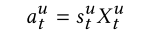aut就表示用户短期意图的表示。为了学习单个注意力表示，我们L自注意表示的均值嵌入作为用户瞬时意图。注意，其他的方式也可以（比如累加啊、求最大啊、最小啊），后面的实验将会比较效果。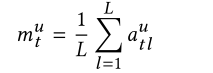输入嵌入的时间信号：以上的自注意力模型不包括时间信号，没有序列信号。输入低级的词袋不能归保留序列模式。我们提议通过位置嵌入提供带有时间信息的query和key。我们使用一个时间尺度的几何序列来向输入端添加不同频率的正弦波。时间嵌入（TE）由以下两个正弦信号组成：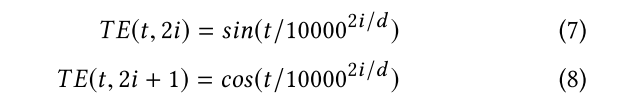其中t是时间步长，i是维度。在非线性变换之前，TE加到query和key里。 整个过程的图如下：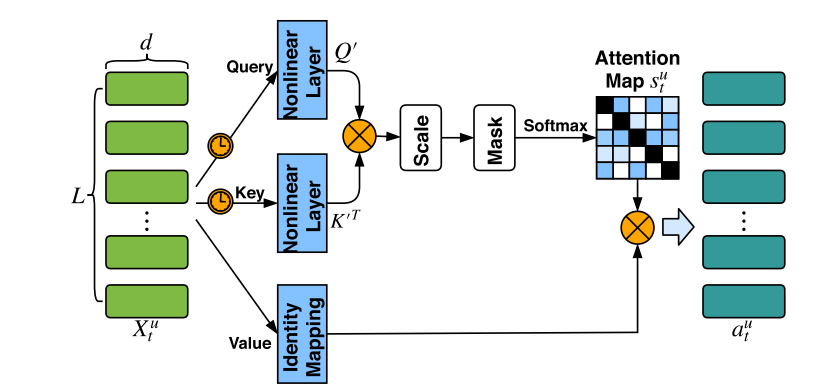3用户长期喜好建模
在对短期效果进行建模之后，将用户的一般品味或长期偏好结合起来是有益的。和潜在因素方法相同，我们为每个用户和每个item分配一个潜在因素。让U属于RMd（Md为上标），V属于RNd（Nd为上标）表明users和item的潜在因素。然而，最新你的研究表明，点积操作违背了度量函数参数不等下的性质，并且将会导致次优解。为了避免这个问题，我们采用欧式距离去测量item i和user u的距离。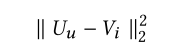越小，距离越近~~~~
4.模型训练
目标函数：给定时间步t的短期意图，和长期偏好。我们的目标是预测用户u在时间t+1下的item（我们用Hu t+1表示，u为上标，t+1为下标）为了保证一致性，我们使用欧式距离，去建模短期和长期的影响。使用其和作为最后的推荐分数。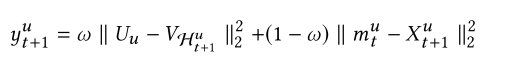上式中，第一项是长期推荐的分数，第二项是短期推荐的分数。注意：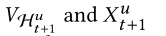都是下一项item的嵌入向量，但是V和U是不一样的参数。最后的分数是由w控制的加权和。 在有些例子中，我们期望去预测多个item。这要求我们的模型，可以捕获序列中的跳跃行为。T+表示用户真正喜欢的下一项T。在我们的论文中，我采用成对排序方法去学习参数，因此我们需要去采样T的反面，即用户不会交互的，不喜欢的item（用T-表示）。显然T-是从I/T+中采样的来的。为了增强消极对和积极对之间的区别。我们使用铰链损失函数：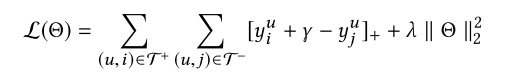上式中，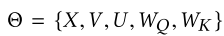是模型参数，r是分离积极和消极对的距离。我们使用l2损失去控制模型的复杂度。DropOut也可以被用于自注意力模型的非线性层。因为我们使用欧式距离，对于稀疏数据集，我们也可以选择范数剪裁策略在欧里几德求中去约束x，u，v。 优化和建议：利用自适应梯度法优化提出得模型，可以自动得适应步长，因此减少学习速率调整所作的努力。
展开全文• 现实世界中的多目标优化问题往往包含不等式约束和等式约束，对于这类带约束条件的多目标优化问题，需要使用有别于无约束优化问题的处理方法。下面首先给出带约束条件的多目标优化问题的的定义： Definition : 约束多...多目标优化 惩罚函数
• 前言Google I/O 2016 上发布了 ConstraintLayout。...关于Cons